## How to Sum/Calculate Total Values with Duplicate Values Only Once in Excel

In statistic when calculating the total values for a range of numbers, we often count duplicate values only once. Normally, if only apply SUM function to calculate total values, it will cover all values including all duplicate values. So, we need to update the formula to make it meet our requirement. This free tutorial will show you the formula to sum values with duplicate values only once in the selected range.

Precondition:

See screenshot below. We want to sum all values but for the duplicate values, we only count once.

## 1. Calculate Total Values with Counting Duplicate Values Only Once using Formula

Step1: In B7, enter the formula:

``=SUMPRODUCT(A2:B6/COUNTIF(A2:B6,A2:B6))``

In this formula, A2:B6 is the range selected for calculating.

Step2: Click Enter to get result. Verify that the sum value is calculated properly. Duplicate values like 100, 60 are only count once.

## 2. Calculate Total Values with Counting Duplicate Values Only Once using User Defined Function

You can create a User-Defined Function (UDF) in VBA to calculate the total values of a range while counting duplicate values only once. Here are the steps of how to do it:

Step1: press ALT + F11 to open the VBA editor in your workbook.

Step2: In the editor, go to Insert > Module to create a new module.

Step3: In the module, paste the following code. Save the module and go back to your Excel workbook.

```Function SumUnique_ExcelHow(ByVal rng As Range) As Double
Dim dict As Object
Set dict = CreateObject("Scripting.Dictionary")

Dim cell As Range
For Each cell In rng
If Not dict.exists(cell.Value) Then
End If
Next cell

Dim key As Variant
For Each key In dict.keys
SumUnique_ExcelHow = SumUnique_ExcelHow + key
Next key
End Function```

Step4: In any blank cell, enter the formula:

``=SumUnique_ExcelHow(A2:B6)``

Where A2:B6 is the range of values you want to calculate the total for.

## 3. Video: Calculate Total Values with Counting Duplicate Values Only Once

This video will show you how to calculate total values with counting duplicate values only once using either the SUMPRODUCT function or a user-defined function with VBA code.

## 4. Related Functions

• Excel SUMPRODUCT function
The Excel SUMPRODUCT function multiplies corresponding components in the given one or more arrays or ranges, and returns the sum of those products.The syntax of the SUMPRODUCT function is as below:= SUMPRODUCT (array1,[array2],…)…
• Excel COUNTIF function
The Excel COUNTIF function will count the number of cells in a range that meet a given criteria. This function can be used to count the different kinds of cells with number, date, text values, blank, non-blanks, or containing specific characters.etc.= COUNTIF (range, criteria)…

## How to Count Cells that do not Contain Errors in Excel

This post will guide you how to count the number of cells that do not contain errors within a range of cells using a formula in Excel 2013/2016 or Excel office 365. How do I count the number of cells without any errors using a simple formula in Excel.

## 1. Count Number of Cells that do Not Contain Errors using Formula

You can use the SUMPRODUCT function in combining with NOT and ISERROR functions to count cells in a specified range of cells that do not contain errors

The below is a generic formula to count the number of cells that do not contain errors:

``=SUMPRODUCT(--NOT(ISERR(range)))``

Supposing that you wish to count the number of cells in range B1:B6 that do not contain errors, and you can use the following formula:

``=SUMPRODUCT(--NOT(ISERR(B1:B6)))``

LET’S SEE THAT HOW THIS FORMULA WORKS:

= NOT(ISERR(B1:B6))

The NOT and ISERROR functions can be used to identify the cells that do not contain an error. And it returns an array result with TRUE or FALSE values. The TRUE values indicate cells without errors, and FALSE values indicate cells with error values.

The array result is like this:

``{TRUE;TRUE;TRUE;FALSE;TRUE;FALSE}``

= –NOT(ISERR(B1:B6))

Then you still need to convert the above array result to their numeric values, 1 and 0, so that the SUMPRODUCT function can calculate the sum of products of corresponding numbers. And you can do this with a double negative operator. And the final array result is like this:

``{1;1;1;0;1;0}``

The SUMPRODUCT function sums the items in the above array and return the final result.

You can also use the SUM function to count the cell that do not contain errors in range B1:B6. And the generic formula is similar with the above formula. It need to be entered as an array formula through pressing `CTRL +SHIFT + Enter` to convert it as array formula. The formula looks like this:

``{=SUM(--NOT(ISERROR(B1:B6)))}``

## 2. Count Cells that do not Contain Errors using User Defined Function with VBA Code

If you want to count the number of cells that do not contain errors in a range, you can write a user defined function with VBA code. Here are the steps to create and run the function:

Step1: Press Alt + F11 keys to open the Microsoft Visual Basic for Applications window.

Step2: Click Insert > Module, and paste the following code in the Module Window.

```Function CountNonErrors_ExcelHow(rng As Range) As Long
Dim cell As Range
Dim count As Long
count = 0
For Each cell In rng
If Not IsError(cell.Value) Then
count = count + 1
End If
Next cell
CountNonErrors_ExcelHow = count
End Function```

Step3: Save and close the window.

Step4: In a blank cell, enter the formula:

``=CountNonErrors_ExcelHow(B1:B6)``

Where B1:B6 is the range you want to count.

Step5: Press Enter to get the result. This function will return the number of cells that do not contain errors in the specified range.

## 3. Video: Count Cells that do not Contain Errors in Excel

This video will show you how to count cells that do not contain errors in Excel using a simple formula and a VBA code.

## 4. Related Functions

• Excel SUMPRODUCT function
The Excel SUMPRODUCT function multiplies corresponding components in the given one or more arrays or ranges, and returns the sum of those products. The syntax of the SUMPRODUCT function is as below:= SUMPRODUCT (array1,[array2],…)…
• Excel ISERROR function
The Excel ISERROR function used to check for any error type that excel generates and it returns TRUE for any error type, and the ISERR function also can be checked for error values except #N/A error, it returns TRUE while the error is #N/A. The syntax of the ISERROR function is as below:= ISERROR (value)….
• Excel SUM function
The Excel SUM function will adds all numbers in a range of cells and returns the sum of these values. You can add individual values, cell references or ranges in excel.The syntax of the SUM function is as below:= SUM(number1,[number2],…)…
• Excel NOT function
The Excel NOT function returns the opposite of a given logical or Boolean value. For example, if you supplied with the value TRUE, the NOT function will return FALSE; If you supplied with the value FALSE, and the NOT function will TRUE. The syntax of the NOT function is as below:=NOT(logical)…

## How to Count Numbers with Leading Zeros

This post will guide you on how to count numbers with leading zeros in Excel 2013/2016/2019/365. When working with numbers that have leading zeros, it can be challenging to count the number of zeros that appear before the actual value. Fortunately, there are two main methods for counting leading zeros in Excel: using a formula or using VBA code.

In this post, we’ll walk through the steps for each method, so you can choose the one that works best for your needs.

## 1. Count Numbers with Leading Zeros using Formulas

Suppost you have a list of product ID in your worksheet, and the product ID contains the different number of leading zeros in the front of string. And if you use the COUNTIF function to count numbers with leading zeros, but it will remove those leading zeros, it means that 1289 and 001289 are the same strings.

So how to preserve the leading zeros while counting numbers. And you can use another Excel function named as SUMPRODUCT.

For example, you want to count strings with leading zeros in the range A2:A6, you can write down the following formula based on the SUMPRODUCT function.

``=SUMPRODUCT(--(\$A\$2:\$A\$6=A2))``

You can enter this formula into Cell B2, then press Enter key. Then you can drag AutoFill Handle down to other cells to apply this formula.

You can also use another excel array formula to count strings with leading zeros as follows:

``=SUM(IF(\$A\$2:\$A\$10=A2,1,0))``

## 2. Count Numbers with Leading Zeros using User Defined Function with VBA Code

Step1: press ALT+F11 to open the VBA editor.

Step2: Click on Insert -> Module to create a new module.

Step3: Copy and paste the following code into the module:

```Function CountLeadingZeros_ExcelHow(cell As Range) As Long
Dim count As Long
Dim value As String
value = cell.value
count = 0
While Left(value, 1) = "0" And Len(value) > 1
count = count + 1
value = Mid(value, 2)
Wend
If Left(value, 1) = "0" Then count = count + 1
End Function```

The VBA code uses a While loop to count the number of leading zero characters in the specified cell. And it checks whether the leftmost character of the cell’s value is “0” and whether the length of the cell’s value is greater than 1.

If both conditions are true, it increments a count variable and removes the leftmost character from the cell’s value using the Mid function.

Step4: Save the module and go back to your Excel worksheet.

Step5: Type the following formula in a blank cell to count the number of leading zero characters in cell A2.

``=CountLeadingZeros_ExcelHow(A2)``

Step6: Press Enter to see the result.

## 3. Video: Count Numbers with Leading Zeros

This video explains how to count numbers with leading zeros in Excel using a formula or VBA code. And it provides step-by-step instructions for both methods and demonstrates how to use them in practice.

## 4. Related Functions

• Excel SUMPRODUCT function
The Excel SUMPRODUCT function multiplies corresponding components in the given one or more arrays or ranges, and returns the sum of those products.The syntax of the SUMPRODUCT function is as below:= SUMPRODUCT (array1,[array2],…)…
• Excel COUNTIF function
The Excel COUNTIF function will count the number of cells in a range that meet a given criteria. This function can be used to count the different kinds of cells with number, date, text values, blank, non-blanks, or containing specific characters.etc.= COUNTIF (range, criteria)…
• Excel IF function
The Excel IF function perform a logical test to return one value if the condition is TRUE and return another value if the condition is FALSE. The IF function is a build-in function in Microsoft Excel and it is categorized as a Logical Function.The syntax of the IF function is as below:= IF (condition, [true_value], [false_value])….
• Excel SUM function
The Excel SUM function will adds all numbers in a range of cells and returns the sum of these values. You can add individual values, cell references or ranges in excel.The syntax of the SUM function is as below:= SUM(number1,[number2],…)…

## 5. Related Posts

• How to insert leading zeros to number or text
Assuming that you want to insert leading zeros  to number in Cell A2, then you can try to use the TEXT function to create an excel formula. You can also use the CONCATENATE function to add the specific digit of leading zeros into each number…
• How to keep or remove leading zeros
If you want to keep the leading zeros as you type in a cell, you need to change the cell format to Text before you type the zip code number.You can remove or delete leading zeros in front of the cells with excel VBA macro…
• Count the number of words in a cell
If you want to count the number of words in a single cell, you can create an excel formula based on the IF function, the LEN function, the TRIM function and the SUBSTITUTE function. ..

## How to Count or Sum Cells with Checked Box in Excel

This post will guide you how to count the number of checked checkboxes in your worksheet in Excel. How do I Count or sum all cells with checked boxes with a formula in Excel.

Assuming that you have a list of data in range A1:C5, in which contain check boxes, product names and sale numbers. And you wish to count the number of all checked boxed in each row in your range. Or you want to sum all sales with checked checkboxes in your range, how to accomplish it. This post will show you one method to sum or count checked checkboxes with the following steps.

## 1. Count or Sum Cells with Checked Box

Step1: you need to select each checked box one by one, and then choose Format Control from the context menu. And the Format Control dialog will open.

Step2: click the Control tab in the Format Control dialog box, and type the cell address in the Cell link text box to link the control to a cell places the value True if the box is checked in the linked cell.

Step3: you need to repeat the above two steps to set a linked cell for other checked boxes.

Step4: then you can insert a formula based on the COUNTIF function in the blank cell that you want to place the last result, such as: Cell E1. Type the following formula into the Cell E1 to Count the number of cells with Checked box in your range A1:C5:

``=COUNTIF(D2:D5,TRUE)``

Then press Enter key on your keyboard to apply this formula. You would see that the number of Cells with checked checkboxes is calculated.

Step5: if you want to sum all sales values with checked checkbox in your range, you can use another formula based on the SUMPRODUCT function to accomplish it. Like this:

``=SUMPRODUCT((\$D\$2:\$D\$5=TRUE)*\$C\$2:\$C\$5)``

Then you need to type this formula in the desired cell, press Enter key on your keyboard to apply it. You would see that the sum of sales value with checked checkboxes is calculated.

## 2. Video: Count or Sum Cells with Checked Box in Excel

In this video, you will learn how to use the COUNTIF and SUMPRODUCT formulas to count or sum cells with checked boxes in Excel.

## 3. Related Functions

• Excel SUMPRODUCT function
The Excel SUMPRODUCT function multiplies corresponding components in the given one or more arrays or ranges, and returns the sum of those products.The syntax of the SUMPRODUCT function is as below:= SUMPRODUCT (array1,[array2],…)…
• Excel COUNTIF function
The Excel COUNTIF function will count the number of cells in a range that meet a given criteria. This function can be used to count the different kinds of cells with number, date, text values, blank, non-blanks, or containing specific characters.etc.= COUNTIF (range, criteria)…

## How to Count the Average Between Two Dates in Excel

In our daily work, we usually have the problem of counting the average of a period. If the given dates are continuous, we can handle this situation very well, and if the dates are discontinuous, how do we count the average for different periods? In this article, we will use some examples to show you how to count the average for two dates by Average formula in different situations.

## 1. Count the Average Between Continuous Dates

First prepare a table with given dates and sales.

You can see the given dates are continuous, so we can count average by Average formula directly.

Step1: In B7, enter the below formula to count the average between dates `1/28/2019` and `3/28/2019`.

``=AVERAGE(B2:B4)``

Step2: Click Enter to get the result.

## 2. Count the Average Between Discontinuous Dates using Formula

If in above table the dates are discontinuous and unordered, how can we count the average of sales? See screenshot below.

You can follow below steps to count the average for two discontinuous dates.

Step1: Prepare another table to save the Start Date, End Date and Average.

Step2: In Start Date enter the start date of the period you want to count the average. In End Date enter the end date of the period you want to count the average. For example, we count the average for period `2/28/2019` and `4/28/2019`.

Step3: In Average, enter the formula:

``=AVERAGE(IF((A2:A7>=B10)*(A2:A7<=B11),B2:B7))``

In above formula, A2:A7 is the given date range, B10 is the start date, B11 is the end date, B2:B7 is the sales range.

Step4: After entering the formula, click `Ctrl + Shift + Enter` to get the result.

Check the formula bar, you can find the formula is displayed as below. That’s an array formula.

Notes:

1. In step 4, if we just click Enter after entering the formula, then we will get an error here. That’s because above formula is an array formula.

2. If we just want to use a normal formula to count the average and click Enter to get the result, we can use below formula:

`` =SUMPRODUCT(--(A2:A7>=B10),--(A2:A7<=B11),B2:B7)/SUMPRODUCT(--(A2:A7>=B10),--(A2:A7<=B11))``

We can get the same result.

## 3. Count the Average Between Discontinuous Dates using User Defined Function with VBA Code

You can also use a User Defined Function with VBA Code to count the average between two given dates in Excel. Just follow these steps:

Step1: Open the Visual Basic Editor (VBE) by pressing Alt + F11 or going to the Developer tab and clicking on Visual Basic.

Step2: Insert a new module by going to the Insert menu and selecting Module.

Step3: Write your user defined function in the module using the Function keyword and the desired name, parameters and return value. Save and close the VBE.

```Function MyAverage_Excelhow(rng1 As Range, rng2 As Range) As Double

Dim sum As Double
Dim count As Long
Dim i As Long
Dim crit1 As Variant
Dim crit2 As Variant

' Check if the ranges have the same size
If rng1.Rows.count <> rng2.Rows.count Or rng1.Columns.count <> rng2.Columns.count Then
MyAverage = CVErr(xlErrValue) ' Return a #VALUE! error
Exit Function
End If

' Get the criteria from cells B10 and B11
crit1 = ActiveSheet.Range("B10").Value
crit2 = ActiveSheet.Range("B11").Value

' Loop through the ranges and calculate the sum and count of the values that meet the criteria
For i = 1 To rng1.Cells.count
If rng1.Cells(i).Value >= crit1 And rng1.Cells(i).Value <= crit2 Then
sum = sum + rng2.Cells(i).Value
count = count + 1
End If
Next i

' Calculate and return the average
MyAverage_Excelhow = sum / count

End Function```

Step4: Go back to Excel and enter a cell where you want to use the function. Type the following formula:

``=MyAverage_Excelhow(A2:A7,B2:B7)``

Step5: press Enter to apply this formula. And the average result will be returned.

## 4. Video: Count the Average Between Two Dates

This video will show you how to count the average between two dates in Excel using a simple formula or a VBA code.

## 5. Related Functions

• Excel SUMPRODUCT function
The Excel SUMPRODUCT function multiplies corresponding components in the given one or more arrays or ranges, and returns the sum of those products.The syntax of the SUMPRODUCT function is as below:= SUMPRODUCT (array1,[array2],…)…
• Excel IF function
The Excel IF function perform a logical test to return one value if the condition is TRUE and return another value if the condition is FALSE. The IF function is a build-in function in Microsoft Excel and it is categorized as a Logical Function.The syntax of the IF function is as below:= IF (condition, [true_value], [false_value])….
• Excel AVERAGE function
The Excel AVERAGE function returns the average of the numbers that you provided.The syntax of the AVERAGE function is as below:=AVERAGE (number1,[number2],…)….

## How to Sum the Smallest N Values in Excel

Sometimes we may want to sum the first smallest N numbers in a range in Excel. In this article, we will show you the method of “SUM the Smallest N Numbers” by a simple formula which consist of SUMPRODUCT and SMALL functions. SMALL can return smallest values based on criteria, SUMPRODUCT can sum up these smallest values.

Through a simple instance, we will introduce you the syntax, argument and the usage of SUMPRODUCT and SMALL functions. We will let you know how the formula works step by step clearly. After reading the article, you may know that in which situations we can choose SUMPRODUCT function to sum data.

## 1. Sum the Smallest N Values Using SUM Formula

In the ‘Value’ column, there are 10 values from ‘1’ to ‘10’ in improper order. Now we want to sum the smallest 4 values from this list, how can we get the correct result?

Actually, we can sort these numbers from smallest to largest firstly by ‘Sort A-Z’ in excel.

Step1: Click ‘Data’ tab, under ‘Sort & Filter’ section click ‘Sort Smallest to Largest’ icon.

Step2: Then you can find numbers are ordered properly.

Step3: Then you can enter SUM function in B2 and select the first four numbers ->cell reference A2:A5 in the list.

Step4: Then you can get the sum of the smallest four values.

## 2. Sum the Smallest N Values Using SUMPRODUCT Formula

We can also get sum conveniently and correctly by just enter a formula. As we want to sum the smallest four numbers from range A2:A11, we can find the smallest N number or numbers by SMALL function actually, and then use SUMPRODUCT function to sum array directly.

Step1: In B2, enter the formula

``=SUMPRODUCT(SMALL(A2:A11,{1,2,3,4}))``

Step2: Press Enter after typing the formula.

We can see that this time we also get correct result 10. The formula works correctly.

HOW THIS FORMULA WORKS

The formula is converted as:

=SUMPRODUCT(SMALL({1;3;5;6;9;10;7;8;4;2},{1,2,3,4}))

Now we will show you how the formula works with the two functions.

For SMALL({1;3;5;6;9;10;7;8;4;2},{1,2,3,4}), refer to k value {1,2,3,4}, we find out the smallest 4 values in the array {1;3;5;6;9;10;7;8;4;2}.

Select SMALL({1;3;5;6;9;10;7;8;4;2},{1,2,3,4}) in the formula bar, press F9. We can get the result {1,2,3,4}.

Obviously, the final result is 1+2+3+4=10. Select SUMPRODUCT({1,2,3,4}) in the formula bar, press F9, 10 is displayed in formula bar.

## 3. Sum the Smallest N Values in Excel with User Defined Function with VBA Code

You can create a user-defined function in Excel to sum the smallest N values in a range of cells. Here are the steps:

Step1: Press Alt + F11 to open the Visual Basic Editor.

Step2: Click Insert > Module to insert a new module.

Step3: Copy and paste the following code into the module. Save the module and close the Visual Basic Editor.

```Function SumSmallestN_Excelhow(rng As Range, n As Integer) As Double
Dim i As Integer
Dim j As Integer
Dim k As Integer
Dim temp As Double
Dim sum As Double

For i = 1 To n
For j = i To rng.Cells.count - 1
If rng.Cells(j).Value < rng.Cells(i).Value Then
temp = rng.Cells(i).Value
rng.Cells(i).Value = rng.Cells(j).Value
rng.Cells(j).Value = temp
End If
Next j
sum = sum + rng.Cells(i).Value
Next i

SumSmallestN_Excelhow = sum
End Function```

Step4: enter the formula into a blank cell:

``=SumSmallestN_Excelhow(A2:A11,4)``

Where A2:A11 is the range of cells you want to sum and 4 is the number of smallest values you want to sum.

Step5: Press Enter to calculate the sum of the smallest 4 values in the range.

## 4. Video: Sum the Smallest N Values in Excel

This video will demonstrate how to sum the smallest N values in Excel using a formula or  a User Defined Function with VBA code as the formula.

## 5. Related Functions

• Excel SUMPRODUCT function
The Excel SUMPRODUCT function multiplies corresponding components in the given one or more arrays or ranges, and returns the sum of those products.The syntax of the SUMPRODUCT function is as below:= SUMPRODUCT (array1,[array2],…)…
• Excel INDIRECT function
The Excel INDIRECT function returns the cell reference based on a text string, such as: type the text string “A2” in B1 cell, it just a text string, so you can use INDIRECT function to convert text string as cell reference….
• Excel ROW function
The Excel ROW function returns the row number of a cell reference.The ROW function is a build-in function in Microsoft Excel and it is categorized as a Lookup and Reference Function.The syntax of the ROW function is as below:= ROW ([reference])….
• Excel SMALL function
The Excel SMALL function returns the smallest numeric value from the numbers that you provided. Or returns the smallest value in the array.The syntax of the SMALL function is as below:=SMALL(array,nth) …
• Excel SUM function
The Excel SUM function will adds all numbers in a range of cells and returns the sum of these values. You can add individual values, cell references or ranges in excel.The syntax of the SUM function is as below:= SUM(number1,[number2],…)

## How to Sum Values Based on Month and Year in Excel

We often do some summary or statistic at the end of one month or one year. In these summary tables, there are at least two columns, one column records the date, and the other column records the sales or product number or total costs etc. If we want to calculate the total values based on a certain month of a year, for example if we want to calculate the total sales for January 2020, how can we do? This article will help you to solve this problem.

See the example below. A column lists some dates. B column lists the sales. E column lists the month and year we want to calculate the total sales for. F column is used for showing the returned value by formula.

## 1. Sum Values Based on Month and Year by SUMIF Function

Step 1: In cell F2, enter the formula:

`` =SUMIFS(B2:B16,A2:A16,">="&DATE(2019,1,1),A2:A16,"<="&DATE(2019,1,31))``

In SUMIFS function, B2:B16 is the sum range, A2:A16 is the criteria range. “>=”&DATE(2019,1,1) and “<=”&DATE(2019,1,31) are the two criteria. Details please see below screenshot.

Verify that total sales 1500 is displayed for January 2019 after calculation.

Step 2: If we want to calculate total sales based on a certain period, we can change the parameters in DATE function. For example, to calculate the total sales for period 1/3/2019 – 1/3/2020, we can enter the formula:

`` =SUMIFS(B2:B16,A2:A16,">="&DATE(2019,1,3),A2:A16,"<="&DATE(2020,1,3))``

Above all, you can change the date in DATE function to do sum per your demands.

## 2. Sum Values Based on Month and Year by SUMPRODUCT

Step 1: In cell F2, enter the formula

``=SUMPRODUCT((MONTH(A2:A16)=1)*(YEAR(A2:A16)=2019)*(B2:B16))``

In this formula we also use MONTH function and YEAR function to filter date from range A2:A16 based on criteria ‘Date is included in period January 2019’. B2:B16 is the sum range.

Step 2: Click Enter to get the result. Verify that we get the same result in method #1 step#1.

## 3. Sum Values Based on Month and Year with User Defined Function (VBA Code)

If you want to sum values based on Month and Year with User Defined Function in Excel, you can use these steps:

Step1: Open the Visual Basic Editor by pressing Alt + F11.

Step2: In the Visual Basic Editor, click on “Insert” from the menu bar and select “Module” to create a new module.

Step3: In the new module, enter the following code, save the module with a suitable name.

```Function SumValuesByMonthAndYear(sumRange As Range, dateRange As Range, sumMonth As Integer, sumYear As Integer) As Double
Dim i As Integer
Dim sum As Double

For i = 1 To sumRange.Rows.Count
If Month(dateRange.Cells(i, 1).Value) = sumMonth And Year(dateRange.Cells(i, 1).Value) = sumYear Then
sum = sum + sumRange.Cells(i, 1).Value
End If
Next i

SumValuesByMonthAndYear = sum

End Function
```

Step4: go back to your Excel worksheet and enter the following formula without the quotes into a blank cell.

``=SumValuesByMonthAndYear(B2:B16, A2:A16, 1, 2019)``

This function takes four arguments:

• sumRange – The range of cells containing the values to sum
• dateRange – The range of cells containing the dates to check for the month and year
• sumMonth – The month to sum for (as an integer from 1 to 12)
• sumYear – The year to sum for (as a four-digit integer)

The function then loops through the dateRange, checks if each date matches the sumMonth and sumYear, and adds the corresponding value from the sumRange to the sum variable.

Finally, the function returns the sum variable as the result.

## 4. Video: Sum Values Based on Month and Year

This video will demonstrate how to Sum Values Based on Month and Year in Excel using the Formula and VBA Code.

## 5. Related Functions

• Excel SUMPRODUCT function
The Excel SUMPRODUCT function multiplies corresponding components in the given one or more arrays or ranges, and returns the sum of those products.The syntax of the SUMPRODUCT function is as below:= SUMPRODUCT (array1,[array2],…)…
• Excel MONTH Function
The Excel MONTH function returns the month of a date represented by a serial number. And the month is an integer number from 1 to 12.The syntax of the MONTH function is as below:=MONTH (serial_number)…
• Excel DATE function
The Excel DATE function returns the serial number for a date.The syntax of the DATE function is as below:= DATE (year, month, day)…
• Excel YEAR function
The Excel YEAR function returns a four-digit year from a given date value, the year is returned as an integer ranging from 1900 to 9999. The syntax of the YEAR function is as below:=YEAR (serial_number)…

## Excel Array Formula

An array formula is a special formula that is converted from a normal formula by the Ctrl+Shift+Enter shortcut.

Excel automatically adds curly brackets “`{}`” at the beginning and end of an array formula. The essence of the array formula is a cell formula, used to explicitly notify the Excel calculation engine to perform multiple calculations on it.

Multiple calculations are the process of performing the relevant calculations separately and simultaneously on the elements of an array that have the corresponding relationships in the formula.

However, not all formulas that perform multiple calculations must be edited into array formulas. Using arrays in the arguments of functions of array type or vector type that return a single result, these functions can automatically perform multiple calculations without using array formulas, such as the SUMPRODUCT function, LOOKUP function, MMULT function and MODE.

## Multi-cell array formula

In a single cell using an array formula for multiple calculations, sometimes you can return a set of results, but the cell can only display a single value (usually the first element of the array results), and can not display the results of the entire group. Using multi-cell array formulas, you can display each element of the result array in a different cell.

### Multi-cell array formula example

Here is a sales table, if you want to calculate the sales volume of different salesmen, then you can multiply the unit price of `E3:E10` by the quantity of `F3:F10`.

The steps are as follows:

STEP1# Select the` G3:G10` cell area, and enter the following formula in the formula bar (excluding the curly brackets on both sides)

``=E3:E10*F3:F10``

STEP2# Press Ctrl+Shift+Enter shortcut keys to convert the formula into an array formula

STEP3# You can see that the sales volume of different salesmen can be calculated by an array formula.

This type of formula that uses the same formula in multiple cells and is converted by the Ctrl+Shift+Enter shortcut is called a “multi-cell array formula“.

The above formula multiplies the unit price of each product by the respective sales quantity to obtain a memory array. The memory array is as below:

``{450;690;250;931;1131;1421;532;1862}``

Then, they are all displayed in the `G3:G10` cell area.

``Note：The curly brackets "{}" at the beginning and end of an array formula are automatically generated by the Ctrl+Shift+Enter shortcut. If you enter curly brackets manually, Excel will recognize them as text characters, and they will not work correctly as formulas.``

## Single-cell array formula

Single-cell array formulas are array formulas that perform multiple calculations in a single cell and return a single value.

### Single-cell array formula example

If you want to calculate the total sales profit of all products, then you can use a single cell array formula to do the statistics.

You can use the following array formula in cell `G12`, and then press Ctrl+Shift+Enter to convert the formula to an array formula.

``=SUM(E3:E10*F3:F10)*G1``

The formula first multiplies the `unit price` and `sales `of each product, and then uses the `SUM function` to add up all the elements in the array to get the `total sales`. Finally, the total sales are multiplied by the `profit margin` in cell `G1`, and the returned value is the `total sales profit `for all products.

As the parameters of the `SUM function` are numeric types, they cannot directly support multiple calculations, so they must be in the form of an array formula to explicitly inform Excel to perform multiple operations.

The formula in this example can be replaced by the `SUMPRODUCT function`:

``=SUMPRODUCT(E3:E10*F3:F10)*G1``

The parameters of the` SUMPRODUCT function` are the array type, which directly supports multiple calculations, so you can enter the formula as a normal formula and get the correct result.

## What is Excel Array?

In Excel functions and formulas, an `array `is a collection of data elements in one row, one column, or multiple rows and columns. Array elements can be numeric, text, date, logical and error values.

The dimension of the array is the direction of the rows and columns of the array. An array with one row and multiple columns is a `horizontal array`, and an array with one column and multiple rows is a `vertical array`. An array with multiple rows and columns has both vertical and horizontal dimensions.

The dimensionality of an array is the number of different dimensions in the array. An array with only one row or column is called a `one-dimensional array`; an array with two dimensions with multiple rows and columns is called a `two-dimensional array`.

The size of an array is expressed by the number of elements in each row and column of the array.

• A `one-dimensional horizontal array` with` 1` rows and `N` columns has a size of `1xN`
• A `one-dimensional vertical array` with `1 `column and `N` rows has a size of `Nx1`
• A `two-dimensional array` with `M` rows and `N` columns has a size of `MxN`

## Excel Array Types

### Constant Array

Constants arrays are string expressions that are written directly to the array elements in a formula and are identified by curly brackets “`{}`” at the beginning and end.

Constant arrays do not depend on the cell range, can be directly involved in the calculation of the formula.

Constant array elements can not be functions, formulas or cell references. Numeric constant elements can not contain dollar signs, commas and percent signs.

#### One-dimensional Array

The elements of a one-dimensional vertical array are separated by a colon “`:`“, the following is an array of numeric constants of size `5x1`.

``={10;20;30;40;50}``

The elements of a one-dimensional horizontal array are separated by a comma “,”, and the following is an array of numeric constants of size 1×5:

``={10,20,30,40,50}``
``Note: For text-based constant arrays, each element in the array is identified by quotation marks by default.``

#### Two-dimensional Array

The elements of a two-dimensional array are separated by a semicolon “`;`” on each row and a comma “`,`” on each column.

The following is a `4×3` two-dimensional array of mixed data types containing numeric, text, date, logical, and error values.

``={10,20,30;"A","B","c";"#N/A!",#REF!,#NUM!;TRUE,FALSE,TRUE}``

The process of manually entering a constant array can be tedious, you can use cell references to simplify the input of constant groups, the steps are as follows:

STEP1# Enter the value of the array element in the cell area, such as `A1:A4`

STEP2# Enter the formula `=A1:A4 `in cell `A5`

STEP3# In the formula bar, select the above formula and press F9, the formula can be converted to a constant array

### Array in Excel Range

Range array is actually a formula directly referenced in the cell range, the size of the array and the size of the constant is exactly the same. For example, the following formulas `A1:A4` and `B1:B4` are range arrays.

``=SUMPRODUCT (A1:A4*B1:B4)``

### Array in Memory

A memory array is an array temporarily formed in memory by multiple values returned by a formula calculation. Memory arrays do not have to be stored in the cell range, and as a group can be directly nested in other formulas to continue to participate in the calculation. For example:

``{ =SMALL(A1:A4,{1,2,3})}``

In the above formula,` {1,2,3} `is a constant group, and the entire formula results in a memory array of `1 `row and `3` columns consisting of the smallest `3` numbers in the range of cells `A1:A4`.

Here is the array in memory.

``={10,20,30}``

The difference between memory array and area array：

• Range array is obtained by cell range reference, memory array is obtained by formula.
• Range Array depends on the referenced cell range, the memory array exists independently in memory.

### Name an array constant

A named array is a constant array, a range array, or a memory array defined using a named formula (i.e., a name) that can be called as an array in a formula.

``Note: You cannot use constant arrays directly in custom formulas used for data validation and conditional formatting, but you can use named arrays created through the Name Manager.``

## Check Cell If Contains One of Many with Exclusions

In Microsoft Excel Spreadsheet or google sheets, when cells contain multiple strings, how can we accomplish this task if you want to check whether these cells contain more than one given string and exclude other given strings?

In this article, we will discuss how to check if a cell contains more than one given string and exclude other given strings by using a formula. You can create a new nested formula by using the SEARCH function (google sheets or Excel), the ISNUMBER function and the SUMPRODUCT function.

The generic formulas are as follows.

`= ( SUMPRODUCT ( -- ISNUMBER (SEARCH(INCLUDE_STRINGS, TEXT_STRING))) > 0 ) * ( SUMPRODUCT ( -- ISNUMBER (SEARCH(EXCLUDE_STRINGS, TEXT_STRING))) = 0 )`

## Check Cell If Contains One of Many with Exclusions in Google Sheets & Excel

Suppose you have two data tables A3:B12, E1:F4, you want to check whether the cell area A3:A12 contains one of the values in the cell range E2:E4, but does not contain any of the values in the cell range F2:F3. You can build the following google sheets or Excel formulas based on the above general formula.

`=(SUMPRODUCT(--ISNUMBER(SEARCH(INCLUDE_STRINGS,A4)))>0) * (SUMPRODUCT(--ISNUMBER(SEARCH(EXCLUDE_STRINGS,A4)))=0)`

where INCLUDE_STRINGS is the named range E2:E4, and EXCLUDE_STRINGS is the named range F2:F3.

You can enter the above formula in cell B4 and press Enter to apply the formula.

Let’s see how this formula works：

= ( SUMPRODUCT ( — ISNUMBER (SEARCH(INCLUDE_STRINGS, A4))) > 0 )

This formula is used to check whether cell A4 contains INCLUDE_STRINGS range of strings, SEARCH function will find from A4 contains INCLUDE_STRINGS range of strings, if it exists, will return the position of the string in cell A4, otherwise return # VALUE!

ISNUMBER formula will SEARCH function return value into TRUE or FALSE, when the search function returns a number, ISNUMBER function will return TRUE, otherwise it will return FALSE.

The double negative sign before ISNUMBER converts the TRUE or FALSE value returned by the ISNUMBER function to 1 and 0.

=(SUMPRODUCT(–ISNUMBER(SEARCH(EXCLUDE_STRINGS,A4)))=0)

This formula is used to check whether the cell does not contain any of the values in F2:F3. Where the SEARCH function is used to perform a lookup string and return the position of the string in cell A4 or return the #VALUE! error message.

## Related Functions

•  Excel ISNUMBER function
The Excel ISNUMBER function returns TRUE if the value in a cell is a numeric value, otherwise it will return FALSE.The syntax of the ISNUMBER function is as below:= ISNUMBER (value)…
• Excel SEARCH function
The Excel SEARCH function returns the number of the starting location of a substring in a text string.The syntax of the SEARCH function is as below:= SEARCH  (find_text, within_text,[start_num])…
The Google Sheets SUMPRODUCT function multiplies corresponding components in the given one or more arrays or ranges, and returns the sum of those products.The syntax of the SUMPRODUCT function is as below:= SUMPRODUCT (array1,[array2],…)…

## Cash Denomination Calculator

Every country has different cash denominations, so you may need to calculate the number of different denominations based on the total amount. If it is just a small amount of cash, then you can calculate the different cash denominations manually, but if multiple cash amounts are needed to calculate the number of denominations, then it is not a good way to do it by manually.

This article will explain how to quickly calculate a given cash denomination value using formulas in a Microsoft Excel spreadsheet or Google Sheets.

## General Formulas for Money Denomination Calculators

In Excel or Google Sheets, you can calculate the cash denomination number by using the following INT function and in combination with the SUMPRODUCT function to create a nested formula as shown below.

`=INT((\$A4-SUMPRODUCT(\$B\$3:B\$3,\$B4:B4))/C\$3)`

For the denomination values in the first column, the following formula needs to be used.

Note: When you execute this formula, you need to make sure that iterative calculation is turned on, otherwise you will see the error message #VALUE!

#### To turn on the Iterative calculation, do the following：

For Microsoft Excel, you need to go to the Excel options window, then go to the Formulas category, and in the Calculation options area, check the box “Enable iterative calculation“. See the following screenshot.

For Google sheets, you need to select the File menu, then click on the Settings submenu, the “Settings for this Spreadsheet” window will pop up, click on the Calculation tab, set the Iterative calculation status to On, and then click on “saving settings“.

### Let’s See How To Use This Formula

STEP 1: Enter the following formula in cell B4 and press Enter to make the formula take effect.

`=INT(\$A4/\$B\$3)`

STEP 2: Select cell B4, drag Fill Handler down to the other cells, so that other cells also apply the formula.

As you can see from the above figure, the value of the first denomination column has been calculated.

STEP 3: Enter the following formula in cell C4 and press Enter to make the formula take effect.

=INT((\$A4-SUMPRODUCT(\$B\$3:B\$3,\$B4:B4))/C\$3)

STEP 4: Select cell C4, drag Fill Handler to the right to the other cells, so that other cells also apply the formula.

STEP 5: Select the cell range C4:G4, drag the Fill Handler on cell G4 down to cell G8, so that other cells also apply the formula.

### Let Me See How This Formula Works

#### =INT(\$A4/\$B\$3)

For the value in column B, since this column is in the first column of the entire denomination column, all we need to do is divide the denomination by Cash Amount, and after that we will remove the decimal part of the returned value by the INT function. For the value of column B will be much easier than other columns, because you do not need to consider the previous counting values.

For example, we can use the following simple formula for the cells in column B.

`=INT(\$A4/\$B\$3)`

#### =SUMPRODUCT(\$B\$3:B\$3,\$B4:B4)

For column C and the subsequent columns, because you need to consider previous count of the denomination column, so you need to sum up the value of all the previous denomination column through the SUMPRODUCT function for a specific column, which contains two arrays, such as \$B\$3: B\$3 used to specify the previous denomination column, \$B4: B4 used to specify the number of denominations have been counted.

Here it should be noted that in the first array, the first cell range reference is absolute reference, the second cell range reference is mixed, so that you can lock a row, while the column will change.

#### =(\$A4-SUMPRODUCT(\$B\$3:B\$3,\$B4:B4))/C\$3

After that, the number of denominations in the current column is calculated by subtracting the original value from the denomination value returned by the SUMPRODUCT function for the previous columns and dividing it by the denomination value in the current column. Finally, the decimal part is removed by the INT function.

### Related Functions

• Excel INT function
The Excel INT function returns the integer portion of a given number. And it will rounds a given number down to the nearest integer.The syntax of the INT function is as below:= INT (number)…
• Excel SUMPRODUCT function
The Excel SUMPRODUCT function multiplies corresponding components in the given one or more arrays or ranges, and returns the sum of those products.The syntax of the SUMPRODUCT function is as below:= SUMPRODUCT (array1,[array2],…)…

## Calculate Win Loss Tie

Suppose you got a task to calculate the win, loss, and tie totals; what would you do? If you are new to Ms Excel and don’t have enough experience with it, then you might do this task manually but let me add that doing this task manually would take a lot of time you will not be able to complete the task at a specific time and you will end up exhausted.

So for calculating a lot of win, loss, and tie totals in a matter of seconds, all you need to do is to read this article thoroughly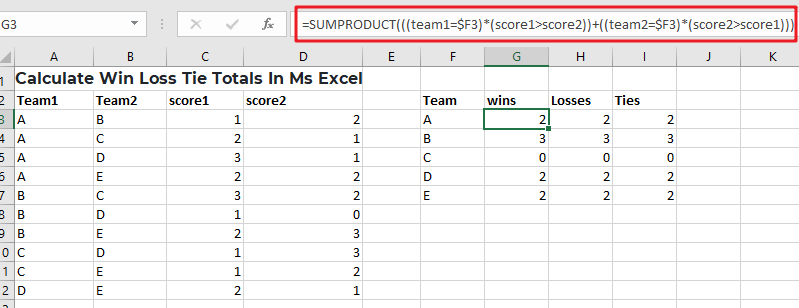So without any further ado, let’s dive into it.

## General Formula

Use the method below to compute win-loss tie totals.

`=SUMPRODUCT(((team1=\$F3)*(score1>score2))+((team2=\$F3)*(score2>score1)))`

## Syntax Explanation

• Parenthesis (): This symbol is used to organize the elements.
• Plus operator (+): This symbol aids in the addition of values.
• SUMPRODUCT: In Excel, this function multiplies the relevant arrays or ranges and returns the total of the products. More information on the SUMPRODUCT Function may be found here.

## Summary

A formula based on the SUMPRODUCT function may be used to determine a team’s win, loss, and tie totals using game data that contains a score for both sides. Type the following formula:

`=SUMPRODUCT(((team1=\$F3)*(score1>score2))+((team2=\$F3)*(score2>score1)))`Based on the data presented, this formula gives total wins for the “A”  team and set the following are the named ranges: team1 (A3:A12), team2 (B3:B12), score1 (C3:C12), and score2 (D3:D12).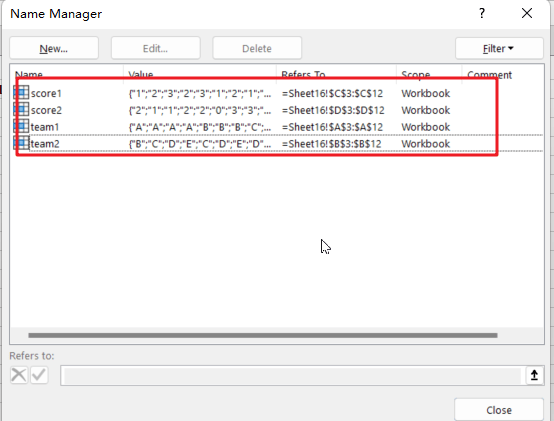### Let’s See How This Formula Works:

In this example, the purpose is to compute total wins, losses, and ties for each team mentioned in column F. The fact that a team can appear in either column A or B complicates matters significantly; therefore, we must account for this when computing wins and losses.

You may consider utilizing the COUNTIF or COUNTIFS function to solve this problem. However, these methods are limited to dealing with established ranges for criteria. Instead, the example formula uses the SUMPRODUCT function to sum the result of a Boolean logic-based array expression. When a team occurs in column A, we use the following equation within SUMPRODUCT on the left:

=((team1=\$F3)*(score1>score2))

This expression comprises two expressions connected by multiplication (*) to construct AND logic.

=(team1=\$F3) / It will verify that if the team is “A”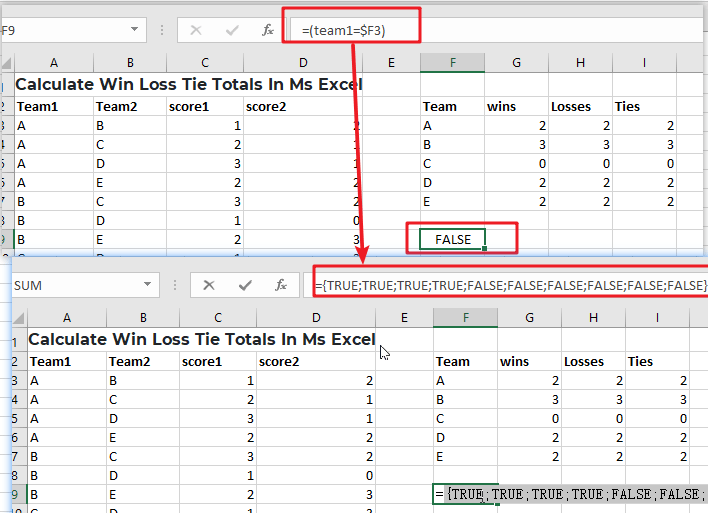The equation on the right determines whether or not the score1 is larger than the score2:

=(score1>score2) / ensure that score1 is larger than the score2.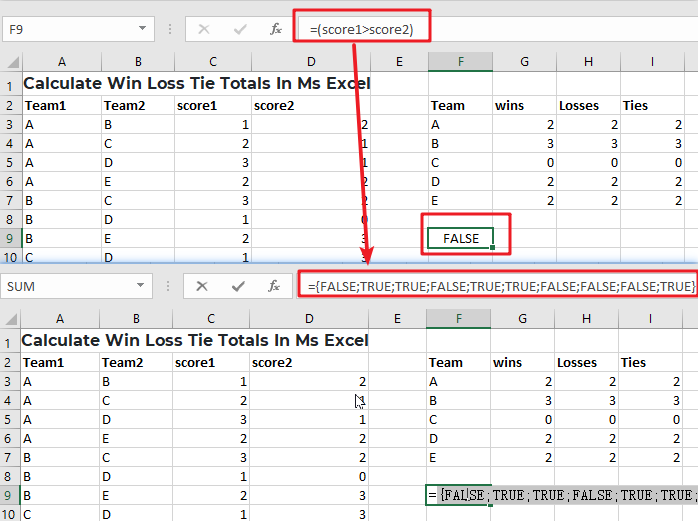Because both expressions utilize multiple-valued ranges, they both yield arrays with numerous outcomes. When we rewrite the formula with the arrays returned.

Multiplication (*) is a math operation that, like AND in Boolean algebra, converts TRUE and FALSE values into 1s and 0s. When both of the related values are TRUE, the outcome is 1. In all other cases, the answer is 0. The end result is a single array that looks like the following: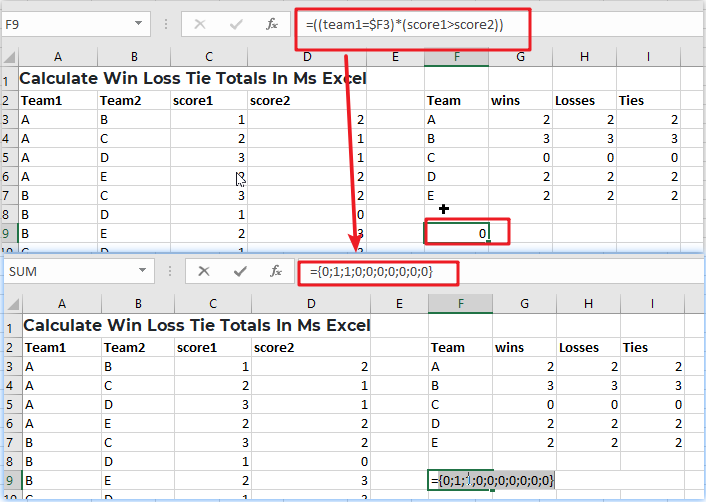We have identical reasoning on the right side that checks for Team A wins when A is Team 2:

=((team2=\$F3)*(score2>score1)))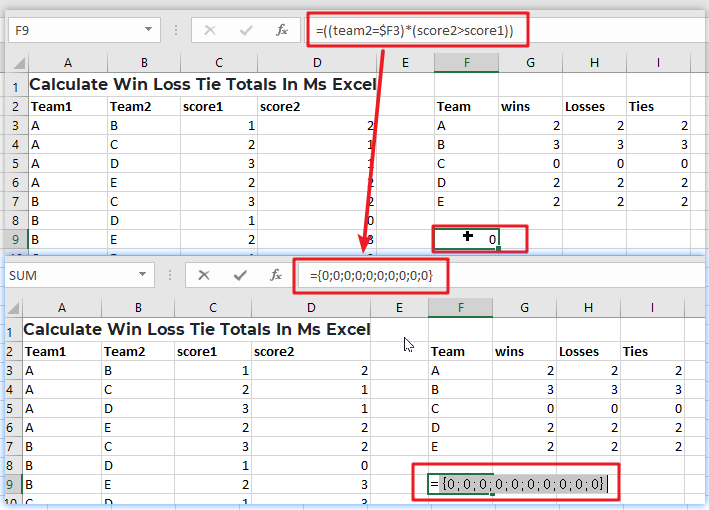Because “A” does not appear as Team 2 in any game, all results are 0.

After evaluating both the left and right sides, we can rewrite the original formula as follows:

`=SUMPRODUCT(({0;1;1;0;0;0;0;0;0;0})+({0;0;0;0;0;0;0;0;0;0}))`

Notice that the arithmetic operator between the two arrays is addition (+), which corresponds to OR logic at this point. We do this because A wins as either Team 1 or Team 2. The result is a single array within SUMPRODUCT:

`=SUMPRODUCT(({0;1;1;0;0;0;0;0;0;0})`

With only one array to process, SUMPRODUCT adds the array’s components and provides a single result, 2.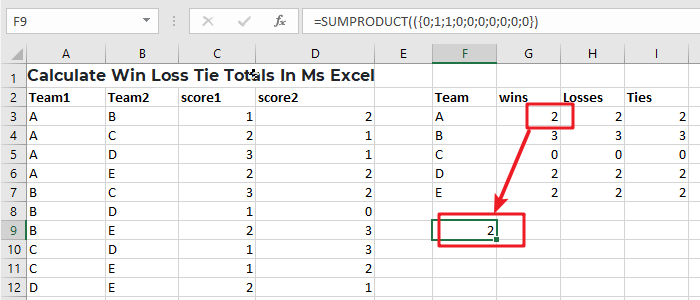### Related Functions

• Excel SUMPRODUCT function
The Excel SUMPRODUCT function multiplies corresponding components in the given one or more arrays or ranges, and returns the sum of those products.The syntax of the SUMPRODUCT function is as below:= SUMPRODUCT (array1,[array2],…)…
• Excel COUNTIF function
The Excel COUNTIF function will count the number of cells in a range that meet a given criteria. This function can be used to count the different kinds of cells with number, date, text values, blank, non-blanks, or containing specific characters.etc.= COUNTIF (range, criteria)…
• Excel COUNTIFS function
The Excel COUNTIFS function returns the count of cells in a range that meet one or more criteria. The syntax of the COUNTIFS function is as below:= COUNTIFS(criteria_range1, criteria1, [criteria_range2, criteria2]…)…

## Count Cells that are Case Sensitive

If you are a frequent user of Microsoft Excel, you may have come across situations where you needed to add or count the cells that were case-sensitive. If you have done this task manually, I would like to point out that it is the most inefficient way to do it, particularly when MS Excel has the versatility to do it in a matter of seconds. As a result of attentively reading this article, you will learn about the many adding or counting case-sensitive cells.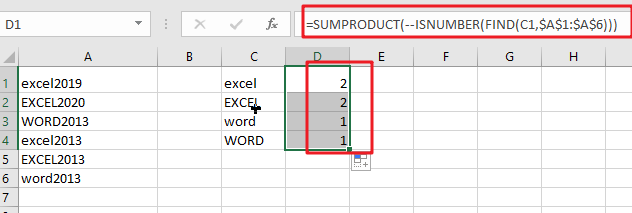Simple generic formula:

```=SUMPRODUCT(--ISNUMBER(FIND(value, range)))
```

## Summary

You may use a formula depending on the ISNUMBER and FIND functions and the SUMPRODUCT function to calculate the number of cells that contain specified text (i.e., hold a substring), taking into consideration upper and lower case. D1 in the example displayed has the following formula, which has been written down:

`=SUMPRODUCT(--ISNUMBER(FIND(C1,\$A\$1:\$A\$6)))`## Let’s See How This Formula Works

In this example, the purpose is to count the number of times each code appears as a substring in a case-sensitive manner, as shown below. When calculating text values, the functions COUNTIF and COUNTIFS are excellent choices. However, since these functions are not case-sensitive, they cannot be utilized to address this issue. To check for substrings, the FIND function should be used in conjunction with the ISNUMBER function, and the SUMPRODUCT function should be used to sum up, the results.

`= FIND(C1,\$A\$1:\$A\$6)`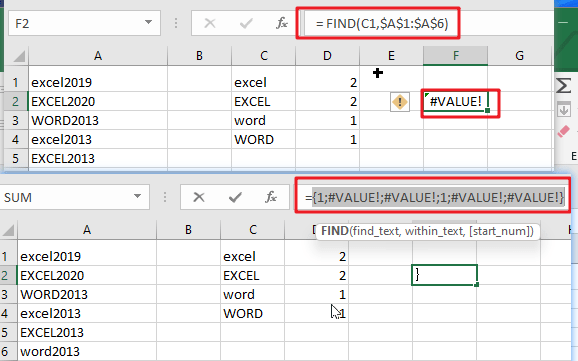The FIND function is case-sensitive and accepts three parameters: find text, within the text, and start num. It is also case-sensitive and takes three arguments. Here is the text we are searching for, and this is the text that we are searching inside. Start num is the number of characters to begin searching in find text. Because start num defaults to 1, we are not supplying a number in this instance. This is necessary since we always want FIND to begin with the first character. When finding the text is discovered inside within text, FIND returns the position of the found text as a number: When finding the text is located within text, FIND returns the position of the found text as a number:

Note: When the find text function fails to yield a result, FIND returns the #VALUE! Error.

`=ISNUMBER(FIND(C1,\$A\$1:\$A\$6))`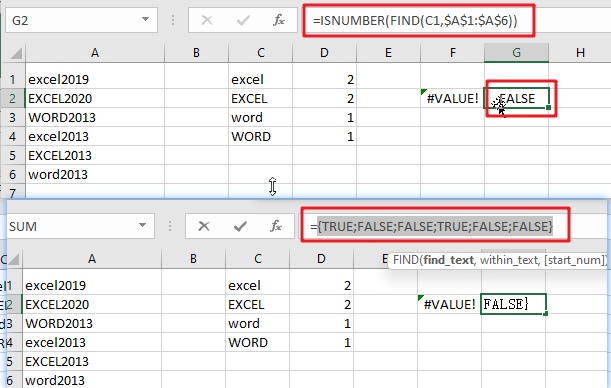This implies that we may be using the ISNUMBER function to transform the output of the FIND function into a TRUE or FALSE value using the ISNUMBER function. A TRUE value will arise from any number, while a FALSE value will result from any error:

As demonstrated in the example, we have four substrings in column C and a variety of codes in columns A1:A6, representing the data for the designated range. Specifically, we want to know how many times each substring in C1:C4 occurs in A1:A6, and we want to know how many times each substring is case-sensitive.

### Related Functions

• Excel SUMPRODUCT function
The Excel SUMPRODUCT function multiplies corresponding components in the given one or more arrays or ranges, and returns the sum of those products.The syntax of the SUMPRODUCT function is as below:= SUMPRODUCT (array1,[array2],…)…
• Excel COUNTIF function
The Excel COUNTIF function will count the number of cells in a range that meet a given criteria. This function can be used to count the different kinds of cells with number, date, text values, blank, non-blanks, or containing specific characters.etc.= COUNTIF (range, criteria)…
• Excel COUNTIFS function
The Excel COUNTIFS function returns the count of cells in a range that meet one or more criteria. The syntax of the COUNTIFS function is as below:= COUNTIFS(criteria_range1, criteria1, [criteria_range2, criteria2]…)…
• Excel ISNUMBER function
The Excel ISNUMBER function returns TRUE if the value in a cell is a numeric value, otherwise it will return FALSE.The syntax of the ISNUMBER function is as below:= ISNUMBER (value)…
• Excel FIND function
The Excel FIND function returns the position of the first text string (sub string) within another text string.The syntax of the FIND function is as below:= FIND(find_text, within_text,[start_num])…

## Check Dates in chronological order

Assume you have a date list that has different date formats, as seen in the accompanying picture. In this instance, Excel’s Sort function will fail to sort them appropriately. However, you may convert all various date formats to a particular format, such as mm/dd/yyy, and then arrange them chronologically. This post will demonstrate how to sort dates in chronological order and how to check if a range of dates are in chronological order.

## Explanation

This is an excellent illustration of how the SUMPRODUCT function may be used to overcome the inability of the COUNTIF or COUNTIFS functions to operate. In this scenario, the purpose is to verify that all dates within a certain range are in chronological order and to display a “YES” value only when dates are in chronological order.

The argument is pretty straightforward, although maybe not apparent. Rather of ensuring that all dates are bigger than the previous one, we determine if any prior date is greater than the next. If we discover even one, the dates will be out of sequence. They are if we discover none (zero).

At its heart, this formula uses the SUMPRODUCT function to determine the number of dates in A1:C1 that are larger than or equal to B1:D1:

`=SUMPRODUCT(--(A1:C1>=B1:D1))`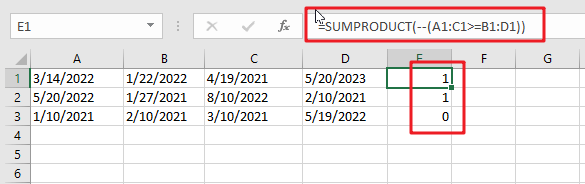Due to the fact that we are employing the larger than or equal to (>=) operator, successive duplicate dates will be disallowed.

This is a two-range operation with 4 dates in each range. As a consequence, an array of TRUE and FALSE values is created as follows: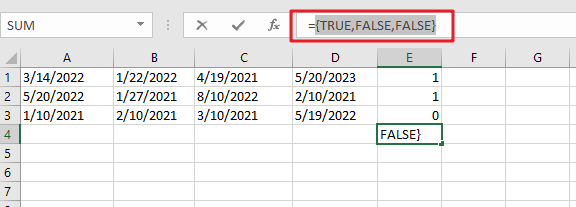You can use the following formula based on the IF function, the SUMPRODUCT function, type:

`=IF(SUMPRODUCT(--(A1:C1>=B1:D1)) =0,”YES”,””)`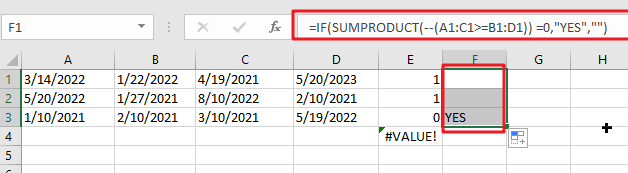In Excel 365, the SORT function offers a convenient alternate solution:

`=IF(SUM(--(A1:D1<>SORT(A1:D1,1,1,1)))=0,"YES","")`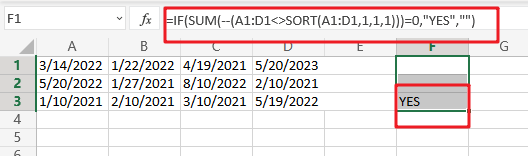The dates in A1:D1 are compared to the same dates following SORT sorting. As with the original algorithm, we are counting any instance when a date is not same (i.e. any date moved by SORT). If there are no dates that vary, a “YES” String Value is returned.

In contrast to the original formula, the SORT version does not check for blank (empty) fields or duplicate dates automatically. The following version has an extra check for blank cells:

`=IF(SUM(((A1:D1<>SORT(A1:D1,1,1,1)))+( A1:D1=""))=0,"YES","")`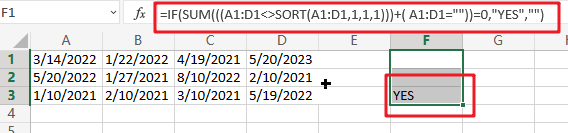In Boolean Algebra, the addition (+) operator behaves similarly to the OR logic. Because the math operation converts TRUE and FALSE values to 1s and 0s automatically, we no longer need the double negative (–).

Note: we use SUM rather than SUMPRODUCT here since Excel 365’s dynamic array support enables SUM to perform array operations natively, without the need for control + shift + enter.

### Related Functions

• Excel SUM function
The Excel SUM function will adds all numbers in a range of cells and returns the sum of these values. You can add individual values, cell references or ranges in excel.The syntax of the SUM function is as below:= SUM(number1,[number2],…)…
• Excel SUMPRODUCT function
The Excel SUMPRODUCT function multiplies corresponding components in the given one or more arrays or ranges, and returns the sum of those products.The syntax of the SUMPRODUCT function is as below:= SUMPRODUCT (array1,[array2],…)…
• Excel COUNTIF function
The Excel COUNTIF function will count the number of cells in a range that meet a given criteria. This function can be used to count the different kinds of cells with number, date, text values, blank, non-blanks, or containing specific characters.etc.= COUNTIF (range, criteria)…
• Excel IF function
The Excel IF function perform a logical test to return one value if the condition is TRUE and return another value if the condition is FALSE. The IF function is a build-in function in Microsoft Excel and it is categorized as a Logical Function.The syntax of the IF function is as below:= IF (condition, [true_value], [false_value])….
• Excel Sort function
The SORT function in Excel sorts the contents of an array or range alphabetically or numerically by columns or rows.The syntax:=SORT(array, [sort index],) …
• Excel COUNTIFS function
The Excel COUNTIFS function returns the count of cells in a range that meet one or more criteria. The syntax of the COUNTIFS function is as below:= COUNTIFS(criteria_range1, criteria1, [criteria_range2, criteria2]…)…

## Case Sensitive Lookup with SUMPRODUCT and EXACT

Today, we will show you how to use SUMPRODUCT and EXACT to perform a case sensitive exact match. In this article, we provide a simple example to calculate bonus for employees whose names are case-sensitive. If you meet similar scenarios in your daily work, you can directly use this formula to deal with your problem.

## EXAMPLE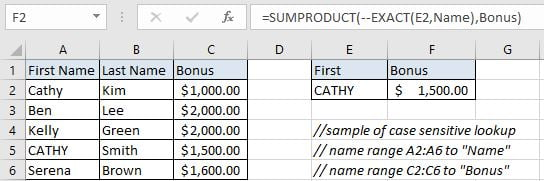In this example, first name “Cathy” and “CATHY” are duplicate but they have different last name. To calculate bonus for “CATHY” correctly, we need to ignore “Cathy” from the list and find out “CATHY” properly, then retrieve bonus “\$1,500.00” from cell C5 properly. To solve this problem, we can create a formula that can perform case-sensitive lookup and retrieves data from a particular position based on the lookup value.

Before creating the formula, to make cell reference and range reference used in the formula are easy to understand, we named range (A2:A6) to “Name”, (C2:C6) to “Bonus”. In this example, we will apply SUMPRODUCT function together with EXACT to perform an exact match.

## ANALYSIS

The formula can perform below operations:

1. It can scan values from “First Name” column and find out lookup value “CATHY” properly.

2. As we have two “Cathy”, we need to ignore the first “Cathy”. Because for the first one, except the first letter, other letters are entered in lowercase. But for our input value “CATHY”, all five letters are entered in UPPERCASE. They are different if case sensitive.

3. After confirming the position of “CATHY”, it can retrieve data from “Bonus” column in the same row of “CATHY”.

4. It returns proper bonus get in step#3 after performing the formula.

Actually, there are some powerful functions and function combinations that can perform lookup effectively and powerfully, for example VLOOKUP function or INDEX/MATCH function combination. However, no matter VLOOKUP function or INDEX/MATCH functions, they are not case-sensitive functions. They will return the first match result ignoring case. So, we need the help from other functions that are case-sensitive and can perform case-sensitive comparison properly. In Excel, EXACT is frequently used for case-sensitive comparison, so, in this example, we will create a formula with EXACT function. At the core, this is a SUMPRODUCT formula, EXACT is applied inside SUMPRODUCT function to perform case-sensitive comparison.

The formula: SUMPRODUCT(–EXACT(E2,Name),Bonus)

## FORMULA

Input formula =SUMPRODUCT(–EXACT(E2,Name),Bonus) into F2. As this is an array formula, so we must press Control+Shift+ENTER to return result. Verify that “\$1,500.00” is displayed properly.## FUNCTION INTRODUCTION

a. SUMPRODUCT function can be seen as SUM+PRODUCT. It returns the sum of products of corresponding ranges or arrays.

Syntax:

`=SUMPRODUCT(array1,array2,array3, ...)`

b. EXACT function is case-sensitive, it can detect whether two strings are exactly the same. If yes, it returns TRUE, else it returns FALSE.

Syntax:

`=EXACT(text1, text2)`

## EXPLANATION

`=SUMPRODUCT(--EXACT(E2,Name),Bonus)`

a. EXACT(E2,Name) performs a case-sensitive comparison between value in E2 (“CATHY”) and values in “Name”({“Cathy”;”Ben”;”Kelly”;”CATHY”;”Serena”}). It returns “TRUE” if the two strings are exactly the same. So, after comparison, we get below an array of “TRUE” and “FALSE”:

`{FALSE;FALSE;FALSE;TRUE;FALSE}`

Select EXACT(E2,Name) in the formula bar and press F9, you can see the result returned by EXACT function: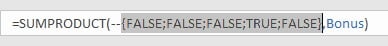b. We add double negative to coerce “TRUE” into number “1” and FALSE into number “0”. Then we get below array consists of numbers “1” and “0”:

`{0;0;0;1;0}`

Select –{FALSE;FALSE;FALSE;TRUE;FALSE} and press F9: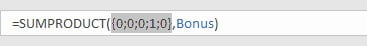c. {0;0;0;1;0} is delivered to SUMPRODUCT function as one array. In this array, “1” represents “CATHY”, its position in the array reflects the position of “CATHY” in range “Name”.

d. Expand values in “Bonus”:

`{1000;2000;2000;1500;1600}`

Select “Bonus” and press F9: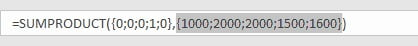SUMPRODUCT multiplies the items in each array and get result {0;0;0;1500;0}; Then returns sum of product: 1500.

## NOTICE

1. SUMPRODUCT only works when items are numeric values in each array. So, in this case, if values in named range “Bonus” are texts, SUMPRODUCT doesn’t work. If you want to retrieve text, you can apply VLOOKUP or INDEX/MATCH with EXACT function.

2. This formula doesn’t work properly if there are multiple matches. It will return the sum of all matches. For example, two “CATHY”.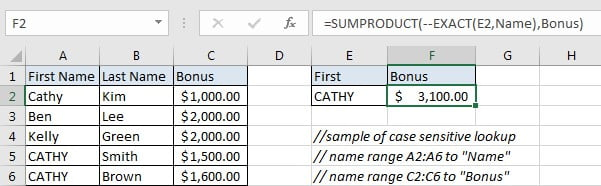### Related Functions

• Excel INDEX function
The Excel INDEX function returns a value from a table based on the index (row number and column number)The INDEX function is a build-in function in Microsoft Excel and it is categorized as a Lookup and Reference Function.The syntax of the INDEX function is as below:= INDEX (array, row_num,[column_num])…
• Excel MATCH  function
The Excel MATCH function search a value in an array and returns the position of that item.The MATCH function is a build-in function in Microsoft Excel and it is categorized as a Lookup and Reference Function.The syntax of the MATCH function is as below:= MATCH  (lookup_value, lookup_array, [match_type])….
• Excel EXACT function
The Excel EXACT function compares if two text strings are the same and returns TRUE if they are the same, Or, it will return FALSE.The syntax of the EXACT function is as below:= EXACT (text1,text2)…
• Excel VLOOKUP function
The Excel VLOOKUP function lookup a value in the first column of the table and return the value in the same row based on index_num position.The syntax of the VLOOKUP function is as below:= VLOOKUP (lookup_value, table_array, column_index_num,[range_lookup])….
• Excel SUMPRODUCT function
The Excel SUMPRODUCT function multiplies corresponding components in the given one or more arrays or ranges, and returns the sum of those products.The syntax of the SUMPRODUCT function is as below:= SUMPRODUCT (array1,[array2],…)…

## Average per Week by Formula in Excel

We usually apply AVERAGE function or relevant functions to return average directly in Excel worksheet. But in some situations, only applying average relevant functions cannot figure out our problem. Sometimes we can create a formula with functions and mathematical operation inside to calculate average depends on different situations.

In this article, through explaining the example below, we will introduce you to apply SUMPRODUCT and COUNTIF functions together to calculate average. You can get the basic knowledge and usage of these two functions in this article.

## EXAMPLE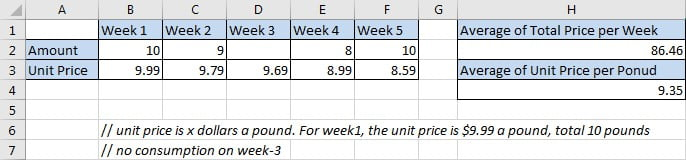The unit for “Amount” is pound. The unit for “Unit Price” is one dollar a pound. From the table we can know that unit prices are fluctuant every week. In different weeks, we bought different amounts of item per our demands. For example, on week-1, we bought 10 pounds with a unit price \$9.99, but on week-3, we didn’t pay for this item. Now, we want to know the average of our consumption per week, and the average of unit price we cost for five weeks.

In this article, to approach our goal, we will apply SUMPRODUCT function and COUNTIF function, and use an expression =SUMPRODUCT()/COUNTIF() to get the average of total price per week; and apply SUMPRODUCT function and SUM function, and expression =SUMPRODUCT()/SUM() to get the average of unit price for one pound during five weeks.

## ANALYSIS

To calculate “average of total price per week”:

a. In mathematics, the general formula to figure out this problem is “Average=(Total price of week1 + Total price of week2 + … + total price of week N)/N weeks”.

b. To calculate the total price of each week, we can create a simple formula that multiplies amount by unit price, for example, for week1, total price is B2*B3.

c The total price of all five weeks is equal to (B2*B3+C2*C3+…+F2*F3). Actually, this operation can be seen as two arrays multiplication that “values from range B2:F2 multiply the values from range B3:F3” correspondingly, then sum all products properly. Till now, we are sure we can apply Excel Math function “SUMPRODUCT” here to sum total price of five weeks simply.

d. For number of weeks, as there is no consumption on week-3, so week-3 should be excluded when counting number of weeks. As D2 is blank, so we can apply COUNT function directly to count weeks as COUNT function can ignore blank cell automatically. To make sense, we apply COUNTIF function instead of COUNT We want to count weeks with consumption, so amount should be greater than 0. To count with one condition or criterion, we choose COUNTIF function here.

e. Then we can create a formula =SUMPRODUCT(B2:F2*B3:F3)/COUNTIF(B2:F2,”>0”) to return average.

To calculate “average of unit price per pound”:

a. In mathematics, the general formula to figure out this problem is “Average=(Total price of week1 + Total price of week2 + … + total price of week N)/Total amounts”. We can repeat above first three steps to get sum of total prices.

b. For total amounts, just apply SUM function which is one of the most frequently used math functions in Excel.

c. Then we can create a formula =SUMPRODUCT(B2:F2*B3:F3)/SUM(B2:F2) to return average.

## FORMULA

Input formula =SUMPRODUCT(B2:F2*B3:F3)/COUNTIF(B2:F2,”>0″) into H2 and press Enter, verify that 86.46 is returned and shown in H2 properly. (10*9.99+9*9.79+8*8.99+10*8.59)/4=86.46. The return value is correct.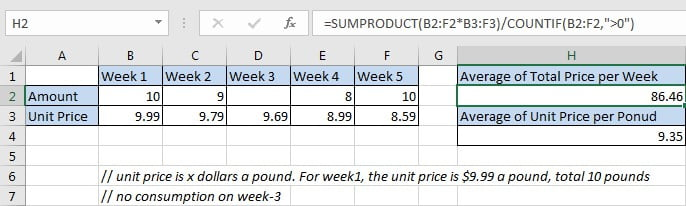Notice: actually, the returned value may be a number with more than two decimal places (based on your settings), you can click “Decrease Decimal” in “Number” section under Home tab to shorten decimal places and keep only two.

Input formula =SUMPRODUCT(B2:F2*B3:F3)/SUM(B2:F2) into H4 and press Enter, verify that 9.35 is returned and shown in H4 properly. (10*9.99+9*9.79+8*8.99+10*8.59)/ (10+9+8+10)=9.35. The return value is correct.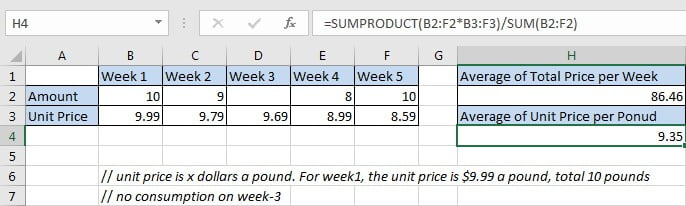## FUNCTION INTRODUCTION

a. SUMPRODUCT function returns the sum of the products of multiplication of multiple corresponding arrays or ranges.

Syntax:

`=SUMPRODUCT(array1, [array2], [array3],…)`

Example.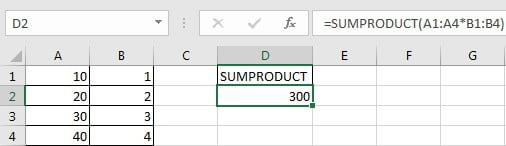b. COUNTIF returns the number of cells which contain a number and the number meets one condition or criterion. The condition or criterion is determined by our input in “criteria” argument.

Syntax:

` =COUNTIF(range, criteria)`

Example.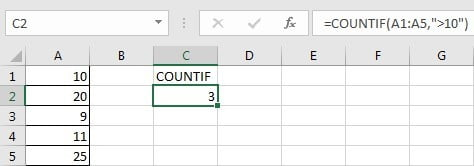c. SUM function returns sum of a set of numbers.

Syntax:

`=SUM(number1,[number2], …)`

Example.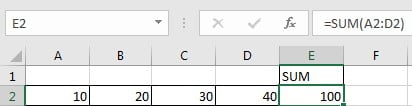## EXPLANATION

`=SUMPRODUCT(B2:F2*B3:F3)/COUNTIF(B2:F2,">0")`

// for SUMPRODUCT, array1 is B2:F2*B3:F3

// for COUNTIF, range is B2:F2, criteria “>0”

a. SUMPRODUCT(B2:F2*B3:F3) returns the sum of total prices of all five weeks. Expand values in B2:F2 and B3:F3, we get below expression:

`=SUMPRODUCT({10,9,0,8,10}*{9.99,9.79,9.69,8.99,8.59}) //`

returns 345.83

b. COUNTIF(B2:F2,”>0″) returns number of weeks with consumption. Expand values from range B2:F2, we get below expression:

`=COUNTIF({10,9,0,8,10},">0")//`

returns “4”

c. Above all, =SUMPRODUCT(B2:F2*B3:F3)/COUNTIF(B2:F2,”>0″) returns 345.83/4=86.46.

`=SUMPRODUCT(B2:F2*B3:F3)/SUM(B2:F2)`

// for SUMPRODUCT, array1 is B2:F2*B3:F3

// for SUM, numbers are saved in B2:F2

a. SUM(B2:F2) returns the sum of total amounts of all five weeks. Expand values in B2:F2, we get below expression:

`=SUM({10,9,0,8,10}) //`

returns 37

b. As SUMPRODUCT function returns 345.83, so =SUMPRODUCT(B2:F2*B3:F3)/COUNTIF(B2:F2,”>0″) returns 345.83/37=9.35.

### Related Functions

• Excel SUM function
The Excel SUM function will adds all numbers in a range of cells and returns the sum of these values. You can add individual values, cell references or ranges in excel.The syntax of the SUM function is as below:= SUM(number1,[number2],…)…
• Excel SUMPRODUCT function
The Excel SUMPRODUCT function multiplies corresponding components in the given one or more arrays or ranges, and returns the sum of those products.The syntax of the SUMPRODUCT function is as below:= SUMPRODUCT (array1,[array2],…)…
• Excel COUNTIF function
The Excel COUNTIF function will count the number of cells in a range that meet a given criteria. This function can be used to count the different kinds of cells with number, date, text values, blank, non-blanks, or containing specific characters.etc.= COUNTIF (range, criteria)…
• Excel AVERAGE function
The Excel AVERAGE function returns the average of the numbers that you provided.The syntax of the AVERAGE function is as below:=AVERAGE (number1,[number2],…)….

## How to Sum if Equal to Many Items or A Range in Excel

If we want to sum numbers from a range with criteria, we often select SUMIF of SUMIFS functions as the first choice to create a formula. The criteria can be a number or an array or a collection of some items. In this article, we will show you to sum numbers based on a range of items. In fact, there are many functions can be applied to help us to figure out this problem, and in real life based on different situations, you can select different functions. But in this article, we will show you the way to resolve this problem by using SUMIF/SUMPRODUCT functions combination. We will introduce you the syntax, arguments and basic usage of these functions, and let you know the working process of our formula.

## EXAMPLE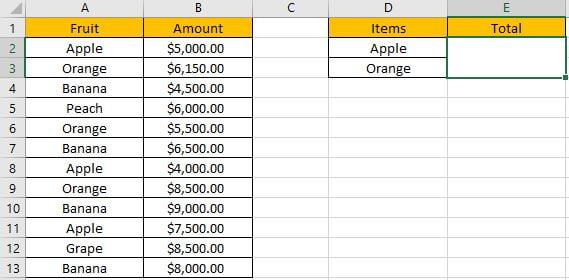Refer to the left-hand side table, we can see some kinds of fruits are listed in “Fruit” column. And amounts are listed in “Amount” column. In the right-hand side table, we list the items we want to sum amounts for them. In previous instance, we have learned how to sum amounts for one item only, for example, we can apply SUMIF of SUMIFS function to create a formula “=SUMIF(A2:A13,D2,B2:B13)” to sum amounts for “Apple”.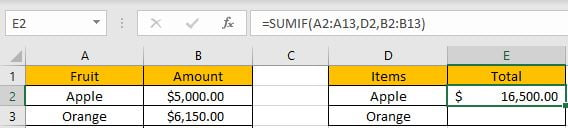But in this case, sum criteria contain two items “Apple” and “Orange”, and the total amount is recorded in one cell and it covers the two items. Thus, only applying SUMIF function cannot figure out our problem, even we can create a formula like “=SUMIF(A2:A13,D2,B2:B13)+SUMIF(A2:A13,D3,B2:B13)” to calculate total amount, it is clear but complex, and when there are multiple items, the formula will be very long. So, we need to find a proper way to sum. In fact, there are many ways to sum numbers based on criteria contain a range reference, for this instance, we will show you to sum amounts for many items by SUMPRODUCT and SUMIF functions.

Before designing a formula, we can name range references.

Select range A2:A13, in name box enter “Fruit”, then press Enter.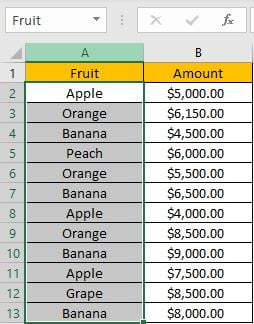Select range B2:B13, in name box enter “Amount”, then press Enter.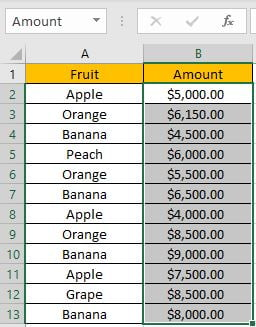Select range D2:D3, in name box enter “Items”, then press Enter.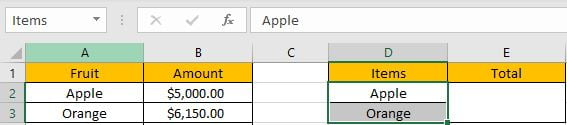## CREATE A FORMULA with SUMPRODUCT & SUMIF FUNCTIONS

### 1.STEPS

Step 1: In E2, enter the =SUMPRODUCT(SUMIF(Fruit,Items,Amount)).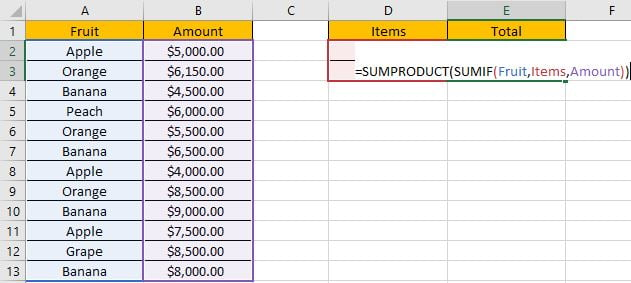You can enter named range like “Fruit” into your formula directly, or you can also select the range by dragging the handle as well.

Step 2: Press Enter after typing the formula.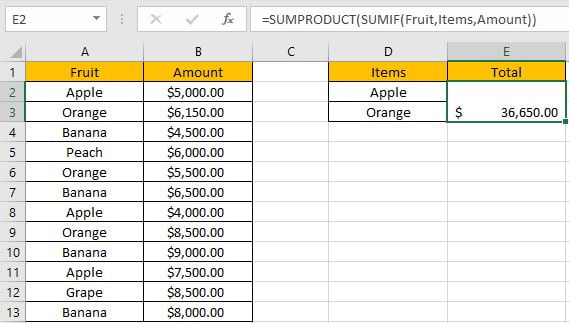We can see in column A, cell A2, A3, A6, A8, A9 and A11 meet our criteria, so we just need to sum numbers from B2, B3, B6, B8, B9 and B11, so the total amount is 5000+6150+5500+4000+8500+7500=36650. The formula works correctly.

### 2.FUNCTION INTRODUCTION

SUMIF function can be seen as SUM+IF. It can sum up numbers by specific condition.

Syntax:

` =SUMIF(criteria_range, criteria, sum_range)`

SUMPRODUCT function can return sum of products.

Syntax:

` =SUMPRODUCT(array1, [array2], [array3], ...)`

For both SUMPRODUCT and SUMIF functions, they support wildcards like asterisk ‘*’ and question mark ‘?’, also support logical operators like ‘>’,’<’. If wildcards or logical operators are required, they should be enclosed into double quotes (““).

### 3.ALL ARGUMENTS

In this case, SUMIF is included in SUMPRODUCT formula as its argument.

SUMIFS – RANGE

Range reference “Fruit” is the criteria range.

In the formula bar, select “Fruit”, press F9, values in this range are expanded in an array.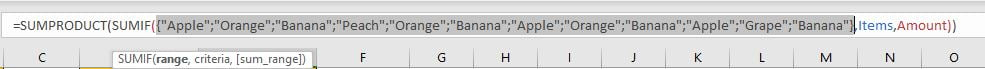SUMIFS – CRITERIA

As we want to sum amount for “Apple” and “Orange” together, so the criteria here is a range reference “Items”.

In the formula bar, select “Items”, press F9, items in this range are expanded in an array.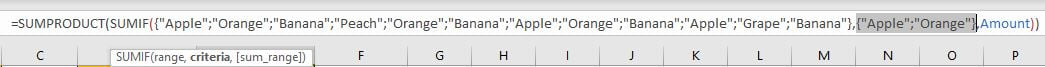As we said we can use “=SUMIF(A2:A13,D2,B2:B13)+SUMIF(A2:A13,D3,B2:B13)” to sum total for both two items, in fact we can use an array constant like {D2;D3} to shorten the formula.

Note: an array constant can provide a set of values, and the values are hard-coded. It is frequently used in array formula.

SUMIF – SUM RANGE

Range reference “Amount” is the “sum range”.

In the formula bar, select ‘Amount’, press F9, amounts in this range are expanded in an array.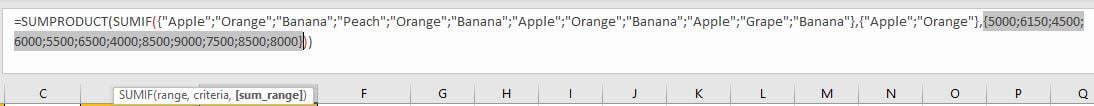### 4.HOW THE FORMULA WORKS

After explaining each argument in the formula, now we will show you how the formula works with these arguments.

After expanding values in each range reference, in the formula bar, the formula is displayed as:

`=SUMPRODUCT(SUMIF({"Apple";"Orange";"Banana";"Peach";"Orange";"Banana";"Apple";"Orange";"Banana";"Apple";"Grape";"Banana"},{"Apple";"Orange"},{5000;6150;4500;6000;5500;6500;4000;8500;9000;7500;8500;8000}))`

A pair of criteria range and criteria:

`{"Apple";"Orange";"Banana";"Peach";"Orange";"Banana";"Apple";"Orange";"Banana";"Apple";"Grape";"Banana"}`

– Criteria Range

{“Apple”;”Orange”} – Criteria (more than one items)

If items from criteria range match either of criteria “Items”, “True” will be recorded in the array, otherwise, “False” will be recorded. So, after comparing with “Apple” and “Orange” separately, the original criteria range is split to two new arrays which only contains “True” and “False”.

{True;False;False;False;False;False;True;False;False;True;False;False} – compare with “Apple”

{False;True;False;False;True;False;False;True;False;False;False;False} – compare with “Orange”

For the following logical operation, “True” is coerced to “1” and “False” is coerced to “0”. So, values in the array are converted to numbers:

{1;0;0;0;0;0;1;0;0;1;0;0} – compare with “Apple”

{0;1;0;0;1;0;0;1;0;0;0;0} – compare with “Orange”

Sum amount of “Apple” refer to sum range:

{1;0;0;0;0;0;1;0;0;1;0;0} – filter “Apple” in criteria range

{5000;6150;4500;6000;5500;6500;4000;8500;9000;7500;8500;8000} – sum_range

Multiply the values from the two arrays in the same position. Then we save the products in an array:

{5000;0;0;0;0;0;4000;0;0;7500;0;0}

Sum amount of “Orange” refer to sum range:

{0;1;0;0;1;0;0;1;0;0;0;0} – filter “Orange” in criteria range

{5000;6150;4500;6000;5500;6500;4000;8500;9000;7500;8500;8000} – sum_range

Multiply the values from the two arrays in the same position. Then we save the products in an array:

{0;6150;0;0;5500;0;0;8500;0;0;0;0}

In this case if we directly select SUMIF part and press F9, array {16500;20150} is returned.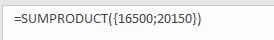We can also use SUM function to replace SUMPRODUCT function in this case.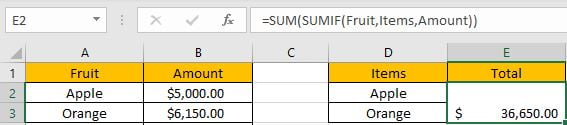## SUMMARY

1. SUMIF function can handle only one pair of criteria range and criteria. Sum range is the last argument among all arguments.

2. SUMPRODUCT function can return the sum of products.

3. They all support wildcards, logical operators.

### Related Functions

• Excel SUMPRODUCT function
The Excel SUMPRODUCT function multiplies corresponding components in the given one or more arrays or ranges, and returns the sum of those products.The syntax of the SUMPRODUCT function is as below:= SUMPRODUCT (array1,[array2],…)…
• Excel SUMIFS Function
The Excel SUMIFS function sum the numbers in the range of cells that meet a single or multiple criteria that you specify. The syntax of the SUMIFS function is as below:=SUMIFS (sum_range, criteria_range1, criteria1, [criteria_range2, criteria2], …)…
• Excel SUMIF Function
The Excel SUMIF function sum the numbers in the range of cells that meet a single criteria that you specify. The syntax of the SUMIF function is as below:=SUMIF (range, criteria, [sum_range])…

## How to Sum if Equal to X or Y in Excel

In daily work, if we want to sum numbers from a range, and only sum the numbers which being equal to X or Y in the range, we can create a formula with Excel build-in functions to get the result. In fact, there are many functions can be applied to help us figure out the problem. In this article, we will show you three different ways to resolve this problem by using SUMIFS/SUMIF/SUMPRODUCT functions. We will introduce you the syntax, arguments and basic usage of these functions, and let you know the working process of different formulas.

## EXAMPLE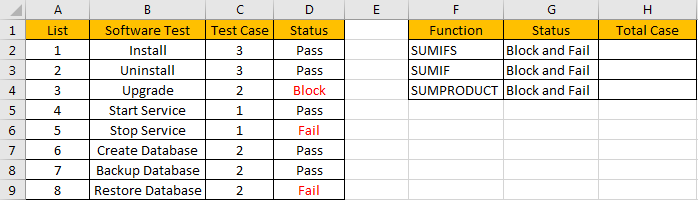Refer to the left-hand side table, we can see some tasks in software test progress are listed, for each task, there are several related test cases, for example, for task “Install”, there are 3 test cases assigned to it. In “Status” column, there are three status “Pass”, “Fail” and “Block”. For the tasks which status is “Fail” or “Block”, we need to re-test the related test cases, so we need to know how many test cases we need to select and execute in next regression test. Now, we need to calculate the re-run test case number. There are many ways to sum numbers based on specific criteria, for this instance, we will show you how to sum numbers by formula with different functions SUMIF/SUMIFS/SUMPRODUCT. We will enter different formulas into H2, H3, H4 of “Total case” column accordingly in the right-hand side table.

Before designing a formula, we can name range references.

Select range C2:C9, in name box enter ‘Case’, then press Enter.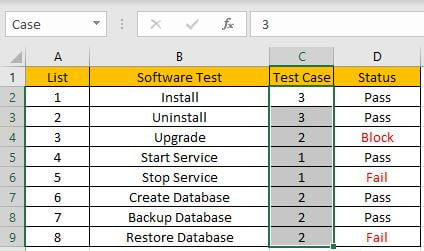Select range D2:D9, in name box enter ‘Status’, then press Enter.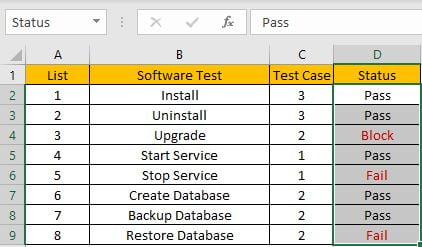## CREATE A FORMULA with SUM & SUMIFS FUNCTIONS

### 1.STEPS

Step 1: In H2, enter the =SUM(SUMIFS(Case,Status,{“Block”,”Fail”})).

`=SUMPRODUCT(C2:C9*(D2:D9={"Block","Fail"}))`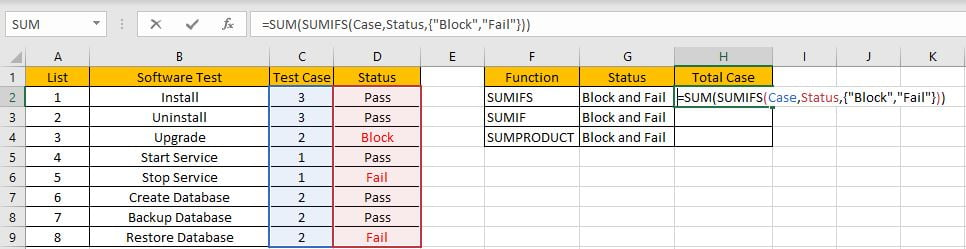Step 2: Press Enter after typing the formula.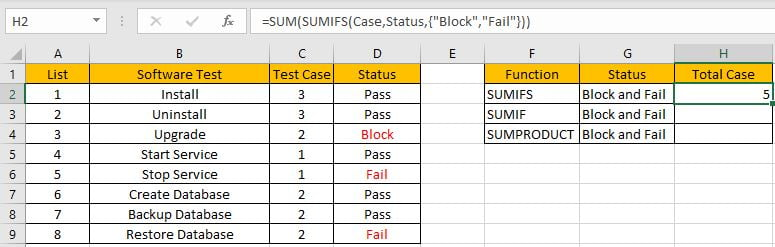We can see in column D, cell D3, D5 and D8 meet our criteria, so we just need to calculate case numbers from C3, C5 and C8, so the total case number is 2+1+2=5. The formula works correctly.

### 2.FUNCTION INTRODUCTION

SUM function can add numbers together. It adds all supplied values from a range or a formula.

Syntax:

`=SUM (number1, [number2], [number3], ...)`

SUMIFS function can be seen as SUM+IFS, it can handle multiple ‘criteria range’ and ‘criteria’ combinations.

Syntax:

`=SUMIFS(sum_range, criteria_range1, criteria1, [criteria_range2, criteria2], ...)`

For both SUM and SUMIFS function, they support wildcards like asterisk ‘*’ and question mark ‘?’, also support logical operators like ‘>’,’<’. If wildcards or logical operators are required, they should be enclosed into double quotes (““).

### 3.ALL ARGUMENTS

In this case, SUMIFS is included in SUM formula, it is the only argument of SUM function, its returned values should be accumulated by SUM function.

SUMIFS – SUM RANGE

In our instance, range reference ‘Case’ is the ‘sum range’. Test case numbers are listed in this field.

In the formula bar, select ‘Case’, press F9, values in this range are expanded in an array.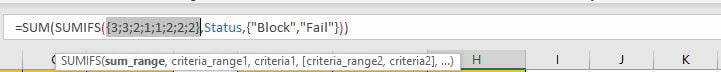SUMIFS – CRITERIA RANGE 1

Range reference ‘Status’ is the criteria range. In this instance we have only one criteria range. We have only three status “Pass”, “Fail” and “Block”.

In the formula bar, select “Status”, pree F9, values in this range are expanded in an array.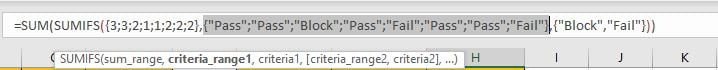SUMIFS – CRITERIA 1

As we want to calculate total test case number for tasks which “status=fail” or “status=block”, so if one condition is matched, test case number will be recorded and accumulated. So, we will supply two criteria “Fail” and “Block” in this case, they are expanded saved in one array, a comma is added between them to split the two criteria. As SUMIFS function supports texts and wildcards, but they should be enclosed into double quotes “”, so for criteria 1, we enter {“Fail”,”Block”}.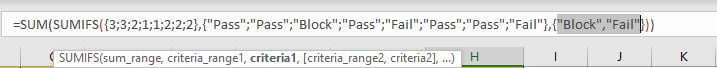Actually, the two criteria can be split to two “criteria1” in two different SUMIFS formulas, for example, if we enter =SUM(SUMIFS(Case,Status,”Fail”),SUMIFS(Case,Status,”Block”)) in H2, we can get the same result.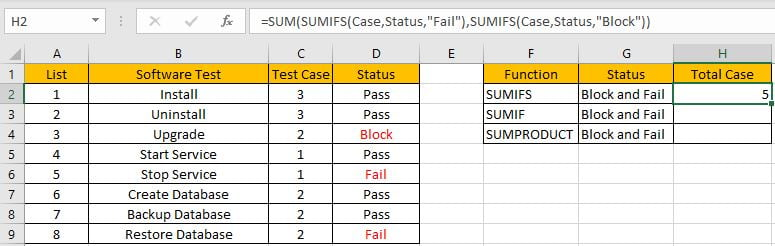This formula is clear but looks a little bit complex, so we use an array constant with two elements to shorten the formula.

### 4.HOW THE FORMULA WORKS

After explaining each argument in the formula, now we will show you how the formula works with these arguments.

After expanding values in each range reference, in the formula bar, the formula is displayed as:

`=SUM(SUMIFS({3;3;2;1;1;2;2;2},{"Pass";"Pass";"Block";"Pass";"Fail";"Pass";"Pass";"Fail"},{"Block","Fail"}))`

If value from the criteria range can match either criteria, “True” will be recorded in this position, otherwise, “False” will be saved instead.

{“Pass”;”Pass”;”Block”;”Pass”;”Fail”;”Pass”;”Pass”;”Fail”} – Criteria Range

{“Block”,”Fail”} – Criteria Collection

So, after comparing, we can get two new arrays based on different criteria:

{False;False;True;False;False;False;False;False} – for criteria “Block”

{False;False;False;False;True;False;False;True} – for criteria “Fail”

For the following logical operation, “True” is coerced to “1” and “False” is coerced to “0”. So above arrays are converted to two arrays only contain numbers “1” and “0”.

{0;0;1;0;0;0;0;0} – for criteria “Block”

{0;0;0;0;1;0;0;1} – for criteria “Fail”

Now, we have below two pairs of arrays:

{3;3;2;1;1;2;2;2} – sum_range

{0;0;1;0;0;0;0;0} – for criteria “Block”

{3;3;2;1;1;2;2;2} – sum_range

{0;0;0;0;1;0;0;1} – for criteria “Fail”

In the two groups, for the elements in the same position, multiply the two elements. Then we can get two new arrays.

{0;0;2;0;0;0;0;0} – for criteria “Block”

{0;0;0;0;1;0;0;2} – for criteria “Fail”

Add all products in above two arrays separately, we get 2 and 3. Actually, if ignore above analysis, directly select SUMIFS part and press F9, a simple array {2,3} is returned.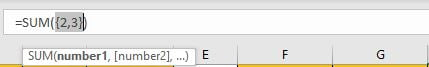Now, the SUM function will add the two numbers together, then we get 5 as last.

## CREATE A FORMULA with SUM & SUMIF FUNCTIONS

SUMIF function is similar with SUMIFS, they have the same arguments, the difference is it can only handle one criteria range with one criterion, and sum range is listed in the end.

`Syntax: =SUMIF(criteria_range, criteria, sum_range)`

In this case, all arguments are the same for SUMIF and SUMIFS, as SUMIF only supports one group of criteria range and criteria, so we need to create two SUMIF formulas, and use SUM function to sum two returned values.

In H3, enter the formula =SUM(SUMIF(Status,{“Block”,”Fail”},Case)).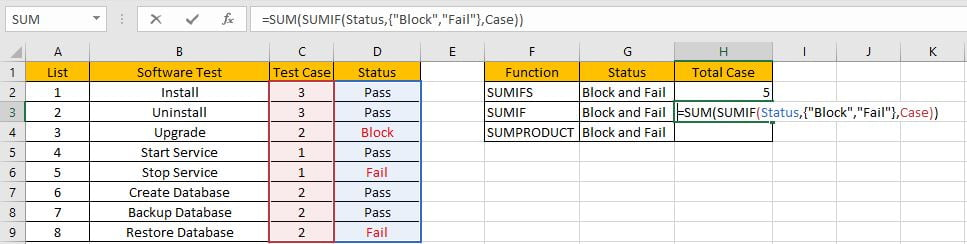Press Enter to get result. We can see we get the same result.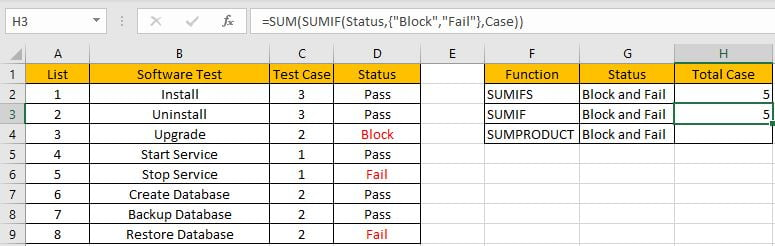## CREATE A FORMULA with SUMPRODUCT FUNCTION

Different with SUMIFS or SUMIF function, SUMPRODUCT function can directly return proper result and ignore SUM function.

### 1.STEPS

Step 1: In H4, enter the =SUMPRODUCT(Case*(Status={“Block”,”Fail”})).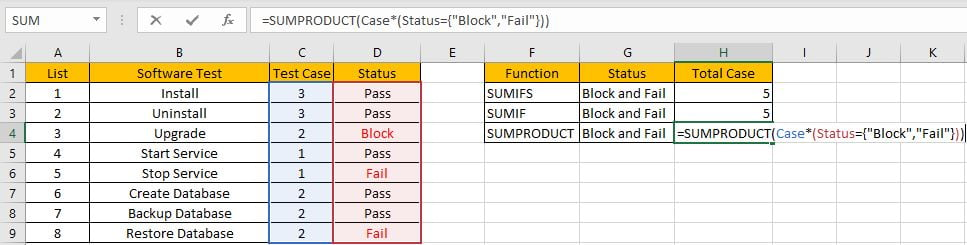Step 2: Press Enter after typing the formula.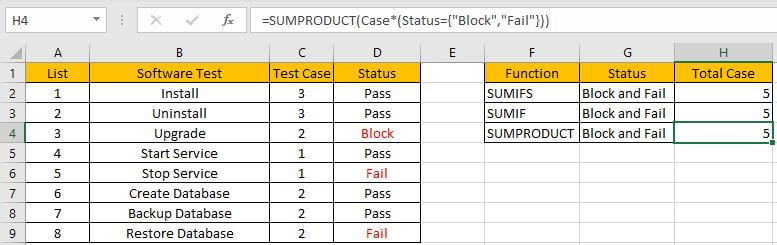### 2.FUNCTION INTRODUCTION

SUMPRODUCT function can be seen as SUM+PRODUCT.

Syntax:

`=SUMPRODUCT(array1, [array2], [array3], ...)`

### 3.ALL ARGUMENTS

SUMPRODUCT – ARRAY1

In this case, we have only one array, it is a formula “Case*(Status={“Block”,”Fail”})”.

In the formula bar, select “Status”, pree F9, values in this range are expanded in an array.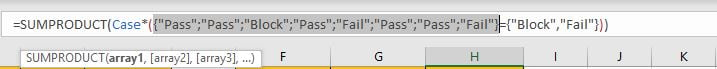Compare each status from range ‘Status’ with the criteria, as there are eight elements in criteria range, and two elements in criteria, so after comparing, system will return a two-dimension array (2*8) in the formula bar.

Just select ({“Pass”;”Pass”;”Block”;”Pass”;”Fail”;”Pass”;”Pass”;”Fail”}={“Block”,”Fail”}), then press F9. Verify that comparative result is displayed.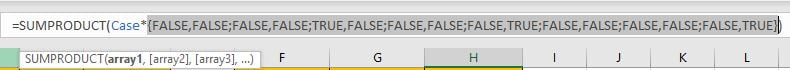Select “Case”, then press F9, all values from range “Case” are expanded.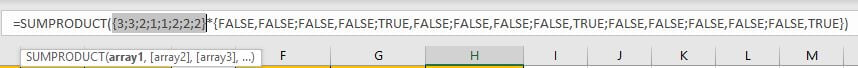### 4.HOW THE FORMULA WORKS

After expanding values in each range reference, in the formula bar, the formula is displayed as:

`=SUMPRODUCT({3;3;2;1;1;2;2;2}*{FALSE,FALSE;FALSE,FALSE;TRUE,FALSE;FALSE,FALSE;FALSE,TRUE;FALSE,FALSE;FALSE,FALSE;FALSE,TRUE})`

Notes: For the following logical operation, “True” is coerced to “1” and “False” is coerced to “0”. The formula is updated to =SUMPRODUCT({3;3;2;1;1;2;2;2}*{0,0;0,0;1,0;0,0;0,1;0,0;0,0;0,1}) in calculation progress.

In the formula bar, select {3;3;2;1;1;2;2;2}*{FALSE,FALSE;FALSE,FALSE;TRUE,FALSE;FALSE,FALSE;FALSE,TRUE;FALSE,FALSE;FALSE,FALSE;FALSE,TRUE} and press F9. We can see that one new array consists of products of previous two arrays are listed.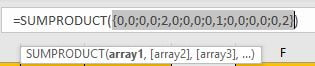Select =SUMPRODUCT({0,0;0,0;2,0;0,0;0,1;0,0;0,0;0,2}), press F9 to do the last step – SUMPRODUCT function will add products in the array together. As 2+1+2=5, so we get 5 by this formula.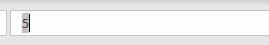SUMMARY

1. SUMIFS function can handle multiple groups of criteria ranges and criteria. Sum range is the first argument among all arguments.

2. SUMIF function can handle only one pair of criteria range and criteria. Sum range is the last argument among all arguments.

3. SUMPRODUCT function can return the sum of products.

4. They all support wildcards, logical operators.

### Related Functions

• Excel SUMPRODUCT function
The Excel SUMPRODUCT function multiplies corresponding components in the given one or more arrays or ranges, and returns the sum of those products.The syntax of the SUMPRODUCT function is as below:= SUMPRODUCT (array1,[array2],…)…
• Excel SUMIFS Function
The Excel SUMIFS function sum the numbers in the range of cells that meet a single or multiple criteria that you specify. The syntax of the SUMIFS function is as below:=SUMIFS (sum_range, criteria_range1, criteria1, [criteria_range2, criteria2], …)…
• Excel SUMIF Function
The Excel SUMIF function sum the numbers in the range of cells that meet a single criteria that you specify. The syntax of the SUMIF function is as below:=SUMIF (range, criteria, [sum_range])…

## How To Sum the Largest N Values in Excel

Sometimes we may want to sum the largest N numbers or top N numbers in a range. In this article, we will show you the method of “SUM the Largest N Numbers” by a simple formula which consist of SUMPRODUCT and LARGE functions. LARGE can return largest values based on criteria, SUMPRODUCT can sum up these largest values.

Through a simple instance, we will introduce you the syntax, argument and the usage of SUMPRODUCT and LARGE functions. We will let you know how the formula works step by step clearly. After reading the article, you may know that in which situations we can choose SUMPRODUCT function to sum data.

## EXAMPLE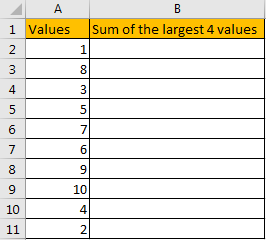In the ‘Values’ column, there are 10 values from ‘1’ to ‘10’ in improper order. Now we want to sum the largest 4 values from this list, how can we get the correct result?

Actually, we can sort these numbers from the largest to the smallest by ‘Sort Z-A’ in excel.

Click ‘Data’ tab, under ‘Sort & Filter’ section click ‘Sort Z to A’ icon.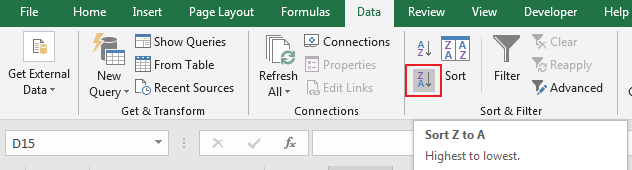Then you can find numbers are ordered properly.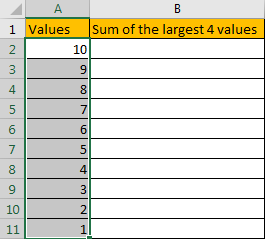Then you can enter SUM function in B2 and select the first four numbers ->cell reference A2:A5 in the list.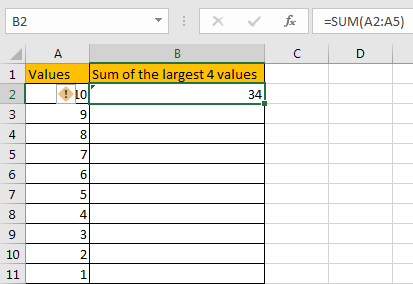Then you can get the sum of the largest four values. It is 34.

In this way, we can get result correctly. But we can also get it conveniently and correctly by just enter a formula. As we want to sum the largest four numbers from range A2:A11, we can find the largest N number or numbers by LARGE function actually, and then use SUMPRODUCT function to sum array directly.

## FORMULA – APPLY SUMPRODUCT FUNCTION

Step 1: In B2, enter the formula

` =SUMPRODUCT(LARGE(A2:A11,{1,2,3,4})).`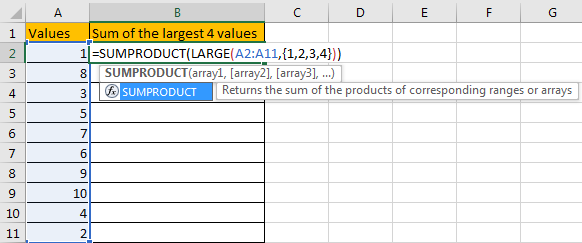Step 2: Press Enter after typing the formula.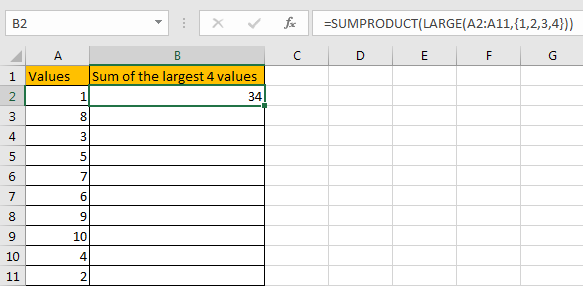We can see that this time we also get correct result 34. The formula works correctly.

## FUNCTION INTRODUCTION

SUMPRODUCT function can be seen as SUM+PRODUCT.

For SUMPRODUCT function, the syntax is:

`=SUMPRODUCT(array1,array2,array3, ...)`

For example, enter =SUMPRODUCT({1,2},{2,3}) in any cell, the we can get value 8, it equals to 1*2+2*3=8. You can also enter =SUMPRODUCT({1,2}*{2,3}), you can get the same result. The argument array can be a real array {1,2,3} for example or a range reference like A2:A7.

If there is only array in the formula, SUMPRODUCT will sum the numbers in the array.

SUMPRODUCT function allows entering texts, logical operators and expressions like (product=cap), texts should be enclosed into ().

LARGE function can return the Nth largest value in a range.

For LARGE function, the syntax is:

`=LARGE(array,k)`

If k is a number, it returns the Kth largest value in the range; if k is an array like {1,2,3}, it returns the largest three values in the range. If k is an array, but the numbers are in inconsecutive sequence for example k={1,2,4}, then LARGE function will return the 1st, 2nd, 4th largest values in the range.

## ARGUMENTS EXPLAINATION

In this formula =SUMPRODUCT(LARGE(A2:A11,{1,2,3,4})), we applied two functions.

For LARGE function, range is A2:A11, k is an array {1,2,3,4}.

Select range A2:A11 in formula bar, press F9 to convert cell reference to real values, numbers in ‘Values’ column are expanded in an array.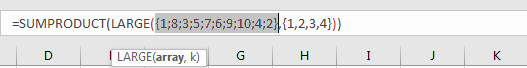For SUMPRODUCT function, there is only one array, it is the result of formula LARGE({1;8;3;5;7;6;9;10;4;2},{1,2,3,4}).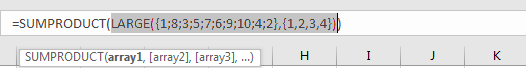## HOW THIS FORMULA WORKS

After explaining argument in the formula, the formula is converted to =SUMPRODUCT(LARGE({1;8;3;5;7;6;9;10;4;2},{1,2,3,4})) in the formula bar. Now we will show you how the formula works with the two functions.

For LARGE({1;8;3;5;7;6;9;10;4;2},{1,2,3,4}), refer to k value {1,2,3,4}, we find out the largest 4 values in the array {1;8;3;5;7;6;9;10;4;2}.

Select LARGE({1;8;3;5;7;6;9;10;4;2},{1,2,3,4}) in the formula bar, press F9. We can get the result {10,9,8,7}. Numbers are ordered from the largest to the smallest.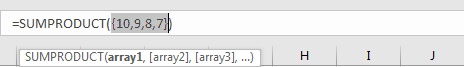Obviously, the final result is 10+9+8+7=34. Select =SUMPRODUCT({10,9,8,7}) in the formula bar, press F9, 34 is displayed in formula bar.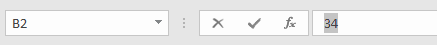1. In this instance, there are only 10 numbers in the range, and we only want to sum the largest 4 numbers. If there are a large amount of numbers, and sum the largest 100 or more numbers in the range, do we need to enter {1,2,3,4,…,100} in LARGE function? Obviously, it is very complex and troublesome. So, we can solve this problem by the help of ROW and INDIRECT

For example, if we want to sum the largest 9 numbers in the same range, we can enter the follow formula in Cell B2:

`=SUMPRODUCT(LARGE(A2:A11,ROW(INDIRECT("1:9"))))`

Then we can get correct value 54.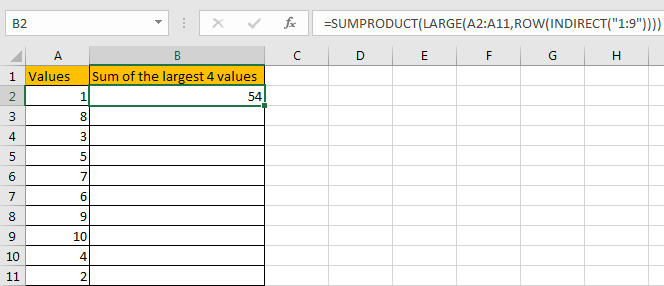In this case, INDIRECT function can return a valid reference based on entered texts. With the help of ROW, ROW(INDIRECT(“1:9”)) can convert “1:9” to {1,2,3,4,5,6,7,8,9}.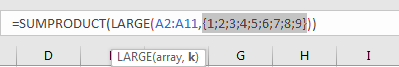2. If N number is saved in another cell, we can concentrate N inside INDIRECT function. Enter the follow formula in Cell d2 this time:

`=SUMPRODUCT(LARGE(A2:A11,ROW(INDIRECT("1:"&D1))))`

See example below: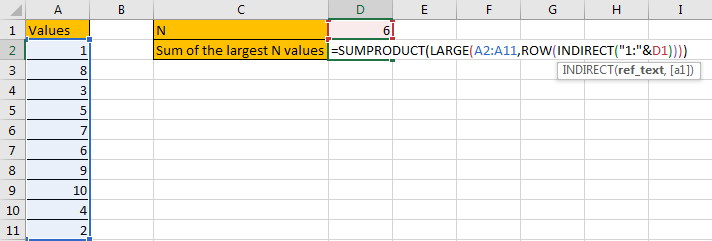In this case, when changing the number in D1, result in D2 will be automatically updated. We don’t need to adjust the formula or cell reference. See example below.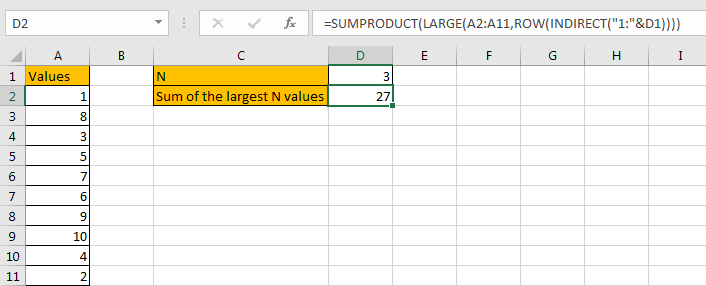3. In this case we can also use SUM function to replace SUMPRODUCT function. But SUMPRODUCT function can handle arrays directly without entering Ctrl + Shift + Enter, so we often apply SUMPRODUCT function in similar situations.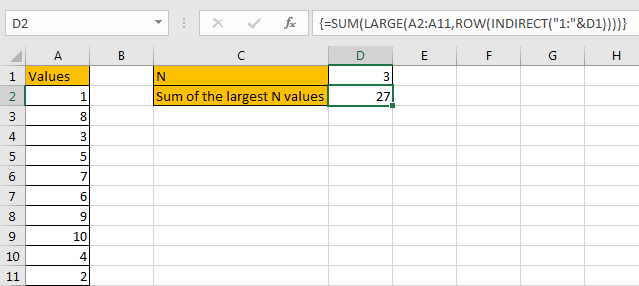SUMMARY

1. SUMPRODUCT function can handle multiple arrays, and it sum up the products.

2. SUMPRODUCT function allows user defined range, wildcards, logical operators, expressions.

3. LARGE function can return the Nth or first Nth largest value in a range.

4. ROW(INDIRECT(“1:N”)) can return a set of numeric numbers in an array.

5. If N is in another cell, use “&” to concentrate them inside INDIRECT, for example ROW(INDIRECT(“1:”&B2)).

### Related Functions

• Excel SUMPRODUCT function
The Excel SUMPRODUCT function multiplies corresponding components in the given one or more arrays or ranges, and returns the sum of those products. The syntax of the SUMPRODUCT function is as below:= SUMPRODUCT (array1,[array2],…)…
• Excel ROW function
The Excel ROW function returns the row number of a cell reference.The ROW function is a build-in function in Microsoft Excel and it is categorized as a Lookup and Reference Function.The syntax of the ROW function is as below:= ROW ([reference])….
• Excel LARGE function
The Excel LARGE function returns the largest numeric value from the numbers that you provided. Or returns the largest value in the array. The syntax of the LARGE function is as below:= LARGE (array,nth)…
• Excel INDIRECT function
The Excel INDIRECT function returns the cell reference based on a text string, such as: type the text string “A2” in B1 cell, it just a text string, so you can use INDIRECT function to convert text string as cell reference….
• Excel SUM function
The Excel SUM function will adds all numbers in a range of cells and returns the sum of these values. You can add individual values, cell references or ranges in excel.The syntax of the SUM function is as below:= SUM(number1,[number2],…)

## How to Sum for Cell Contains Formula Only in Excel

Sometimes values are created by formulas in cells. If we want to sum values which are created by formulas from a range, but some values which are hardcoded also list in the same range, how can we filter out matched data and only sum the filtered values correctly? By the way, if we want to sum cells that contains formulas, how can we do? Actually, we can apply ISFORMULA function to help us to solve our problem, it can return true of false if call contains a formula or not.

In this article, we will show you the method of “SUM for Cells that Contains Formula” with the application of ISFORMULA and SUMPRODUCT functions. We will create a simple formula which consists of the two functions.

We will provide a very simple instance in this article, and we will show you the syntax, argument and the usage of ISFORMULA and SUMPRODUCT functions. We will let you know how the formula works step by step clearly. After reading the article, you may know that in which situations we can choose SUMPRODUCT function to sum data.

## EXAMPLE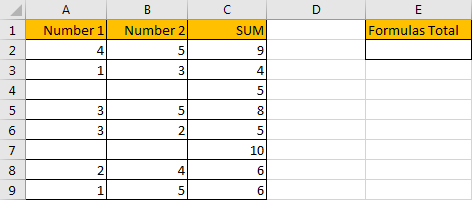In above table, we list the sum of two numbers in SUM column. But for some other numbers in the same column, they are hardcoded for example 5 in C4 and 10 in C7. In E column, we want to sum numbers which are created by formula. If we want to sum numbers ignore the hardcoded numbers, we need the help of ISFORMULA function, it can distinguish if number is generated by a formula or not. Then after filtering numbers, we can apply SUMPRODUCT function to sum filtered numbers.

## FORMULA – APPLY SUMPRODUCT FUNCTION

Step 1: In E2, enter the formula

`=SUMPRODUCT(C2:C9*ISFORMULA(C2:C9))`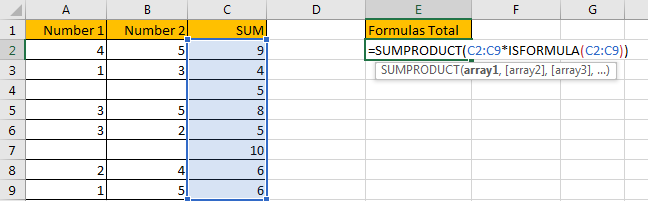Step 2: Press Enter after typing the formula.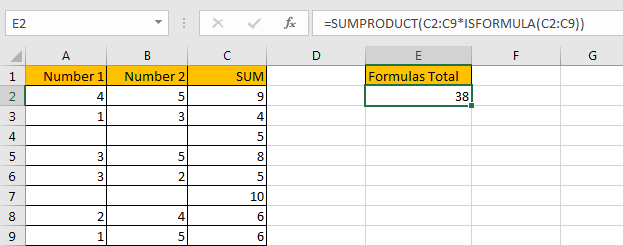We can see that the result is 38, it equals to 9+4+8+5+6+6=38. The formula works correctly.

## FUNCTION INTRODUCTION

SUMPRODUCT function can be seen as SUM+PRODUCT.

For SUMPRODUCT function, the syntax is:

`=SUMPRODUCT(array1,array2,array3, ...)`

For example, enter =SUMPRODUCT({1,2},{2,3}) in any cell, the we can get value 8, it equals to 1*2+2*3=8. You can also enter =SUMPRODUCT({1,2}*{2,3}), you can get the same result. The argument array can be a real array {1,2,3} for example or a range reference like C2:C7.

If there is only array in the formula, SUMPRODUCT will sum the numbers in the array.

Besides, SUMPRODUCT function allows entering texts, logical operators and expressions like (product=cap), texts should be enclosed into ().

ISFORMULA function can return True if cell contains a formula, or if a number is created by a formula; if returns False, that means this cell doesn’t contain any formula.

For ISFORMULA function, the syntax is:

`=ISFORMULA (reference)`

## ARGUMENTS EXPLAINATION

In this formula =SUMPRODUCT(C2:C9*ISFORMULA(C2:C9)), we applied two functions.

For ISFORMULA function, reference is C2:C9.

For SUMPRODUCT function, there is only one array argument, it is C2:C9*ISFORMULA(C2:C9).

## HOW THIS FORMULA WORKS

After explaining argument in the formula, let’s start to execute this formula from inside to outside.

First, in the formula bar, we execute ISFORMULA part. Select ISFORMULA(C2:C9), press F9 to get result, we found that an array is returned. True and False are saved in this array.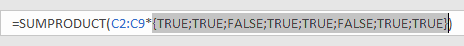Then select C2:C9 in the formula bar, press F9. We can see that values in C2:C9 are expanded in this array.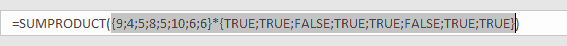Actually, in excel, if the two arrays are multiplied by each other, TRUE will be forced to convert to 1 and FALSE will be converted to 0 in math operation. So, {TRUE;TRUE;FALSE;TRUE;TRUE;FALSE;TRUE;TRUE} will be converted to {1,1,0,1,1,0,1,1} in next step of calculation. At last, this formula can be seen as

`=SUMPRODUCT({9;4;5;8;5;10;6;6}*{1,1,0,1,1,0,1,1}).`

As SUMPRODUCT function can sum products from arrays. That’s why in this case we apply SUMPRODUCT function instead of other sum related functions.

Continue executing the formula. Select {9;4;5;8;5;10;6;6}*{TRUE;TRUE;FALSE;TRUE;TRUE;FALSE;TRUE;TRUE} in the formula bar, press F9. Let’s see if products from above two arrays are displayed.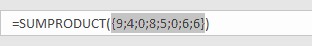As we can see, products are saved a new array {9;4;0;8;5;0;6;6}.

Select =SUMPRODUCT({9;4;0;8;5;0;6;6}) in the formula bar, press F9, 38 is displayed in formula bar. 38 is the final result.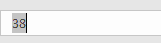1. In this instance, if we want to sum cells which doesn’t contain any cell, we can add NOT before ISFORMULA

Enter the follow formula in Cell E2:

` =SUMPRODUCT(C2:C9*NOT(ISFORMULA(C2:C9)))`

We can get result 15.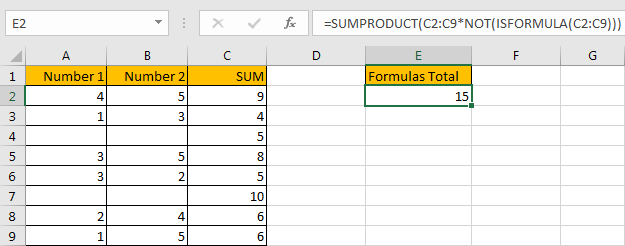NOT function here can return the opposite of the given formula ISFORMULA(C2:C9).

2. In this case we can also use SUM function to replace SUMPRODUCT function. But SUMPRODUCT function can handle arrays directly without entering Ctrl + Shift + Enter, so we often apply SUMPRODUCT function in similar situations.

After applying SUM function, press Ctrl + Shift + Enter simultaneously, we can also get correct result 38.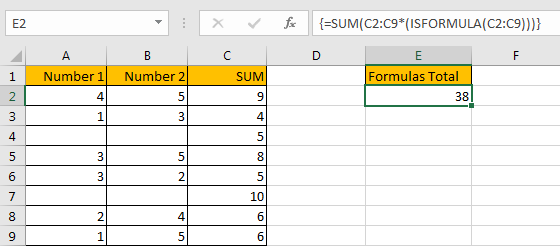## SUMMARY

1. SUMPRODUCT function can handle multiple arrays, and it sum up the products.

2. SUMPRODUCT function allows user defined range, wildcards, logical operators, expressions.

3. ISFORMULA function can return True/False if cell contains a formula or not.

4. NOT function can return the opposite of a given formula or logical operation in excel.

## Related Functions

• Excel SUMPRODUCT function
The Excel SUMPRODUCT function multiplies corresponding components in the given one or more arrays or ranges, and returns the sum of those products.The syntax of the SUMPRODUCT function is as below:= SUMPRODUCT (array1,[array2],…)…
• Excel SUM function
The Excel SUM function will adds all numbers in a range of cells and returns the sum of these values. You can add individual values, cell references or ranges in excel.The syntax of the SUM function is as below:= SUM(number1,[number2],…)
• Excel NOT function
The Excel NOT function returns the opposite of a given logical or Boolean value. For example, if you supplied with the value TRUE, the NOT function will return FALSE; If you supplied with the value FALSE, and the NOT function will TRUE. The syntax of the NOT function is as below:=NOT(logical)…